# US6115188A - Optical system and optical apparatus having the same - Google Patents

## Info

Publication number
US6115188A
US6115188A US09/172,164 US17216498A US6115188A US 6115188 A US6115188 A US 6115188A US 17216498 A US17216498 A US 17216498A US 6115188 A US6115188 A US 6115188A
Authority
US
United States
Prior art keywords
lens
optical
subunit
image
positive
Prior art date
Legal status (The legal status is an assumption and is not a legal conclusion. Google has not performed a legal analysis and makes no representation as to the accuracy of the status listed.)
Application number
US09/172,164
Inventor
Akihiro Nishio
Hideki Ogawa
Makoto Misaka
Current Assignee (The listed assignees may be inaccurate. Google has not performed a legal analysis and makes no representation or warranty as to the accuracy of the list.)
Canon Inc
Original Assignee
Canon Inc
Priority date (The priority date is an assumption and is not a legal conclusion. Google has not performed a legal analysis and makes no representation as to the accuracy of the date listed.)
Filing date
Publication date
Priority to JP29957397A priority Critical patent/JP3486541B2/en
Priority to JP9-299573 priority
Priority to JP34585997A priority patent/JP3745104B2/en
Priority to JP9-345859 priority
Priority to JP27661898A priority patent/JP4272725B2/en
Priority to JP10-276618 priority
Application filed by Canon Inc filed Critical Canon Inc
Assigned to CANON KABUSHIKI KAISHA reassignment CANON KABUSHIKI KAISHA ASSIGNMENT OF ASSIGNORS INTEREST (SEE DOCUMENT FOR DETAILS). Assignors: MISAKA, MAKOTO, OGAWA, HIDEKI, NISHIO, AKIHIRO
Application granted granted Critical
Publication of US6115188A publication Critical patent/US6115188A/en
Anticipated expiration legal-status Critical
Expired - Lifetime legal-status Critical Current

• 230000003287 optical Effects 0.000 title claims abstract description 155
• 230000000694 effects Effects 0.000 claims abstract description 13
• 239000003570 air Substances 0.000 claims description 22
• 239000000463 materials Substances 0.000 claims description 13
• 230000005499 meniscus Effects 0.000 claims description 9
• 230000004075 alteration Effects 0.000 description 129
• 230000000875 corresponding Effects 0.000 description 21
• 230000000087 stabilizing Effects 0.000 description 21
• 238000006073 displacement reactions Methods 0.000 description 16
• 101000444290 Saccharomyces cerevisiae (strain ATCC 204508 / S288c) Transposon Ty3-I Gag-Pol polyprotein Proteins 0.000 description 13
• 101000488238 Saccharomyces cerevisiae (strain ATCC 204508 / S288c) Chromatin structure-remodeling complex subunit RSC4 Proteins 0.000 description 11
• 101000006069 mouse Cystic fibrosis transmembrane conductance regulator Proteins 0.000 description 8
• 230000035945 sensitivity Effects 0.000 description 8
• 101000534602 Dengue virus type 2 (strain 16681-PDK53) Genome polyprotein Proteins 0.000 description 7
• 101000182274 mouse Ig gamma-2A chain C region, A allele Proteins 0.000 description 7
• 101000524125 mouse Ig kappa chain V-III region PC 3741/TEPC 111 Proteins 0.000 description 7
• 101000193954 Aplysia californica ADP-ribosyl cyclase/cyclic ADP-ribose hydrolase Proteins 0.000 description 6
• 210000000188 Diaphragm Anatomy 0.000 description 6
• 210000000554 Iris Anatomy 0.000 description 6
• 238000000926 separation method Methods 0.000 description 6
• 239000011521 glasses Substances 0.000 description 5
• 101000103661 human Tumor necrosis factor ligand superfamily member 18 Proteins 0.000 description 5
• 206010010071 Coma Diseases 0.000 description 4
• 101000446889 human Aldo-keto reductase family 1 member B1 Proteins 0.000 description 4
• 101000396470 human COP9 signalosome complex subunit 6 Proteins 0.000 description 4
• 101000466095 human Lysine-specific histone demethylase 1B Proteins 0.000 description 4
• 101000518475 human Putative oxidoreductase GLYR1 Proteins 0.000 description 4
• 101000072365 xenopus laevis Histone H2A type 1 Proteins 0.000 description 4
• 101000240322 xenopus laevis Histone H2B 1.1 Proteins 0.000 description 4
• 101000405401 xenopus laevis Histone H3.2 Proteins 0.000 description 4
• 101000351967 xenopus laevis Histone H4 Proteins 0.000 description 4
• 101000162978 Pyrococcus horikoshii (strain ATCC 700860 / DSM 12428 / JCM 9974 / NBRC 100139 / OT-3) UDP-N-acetyl-D-mannosamine dehydrogenase Proteins 0.000 description 3
• 101000433371 Saccharomyces cerevisiae (strain ATCC 204508 / S288c) Osmosensing histidine protein kinase SLN1 Proteins 0.000 description 3
• 101000166924 Saccharomyces cerevisiae (strain ATCC 204508 / S288c) Phosphorelay intermediate protein YPD1 Proteins 0.000 description 3
• 101000546486 Staphylococcus epidermidis Serine-aspartate repeat-containing protein G Proteins 0.000 description 3
• 238000010586 diagrams Methods 0.000 description 3
• 239000005357 flat glasses Substances 0.000 description 3
• 101000171581 human Aurora kinase A Proteins 0.000 description 3
• 101000005841 human Cyclin-dependent kinase 2 Proteins 0.000 description 3
• 101000433555 human Superoxide dismutase [Cu-Zn] Proteins 0.000 description 3
• 239000010950 nickel Substances 0.000 description 3
• 101000473476 Bacillus subtilis (strain 168) Nitrogen regulatory PII-like protein Proteins 0.000 description 2
• 101000255158 Enterobacteria phage T7 Portal protein Proteins 0.000 description 2
• 101000495746 Enterobacteria phage T7 Tail tubular protein gp11 Proteins 0.000 description 2
• 101000495747 Enterobacteria phage T7 Tail tubular protein gp12 Proteins 0.000 description 2
• 101000506971 Escherichia coli (strain K12) Biotin synthase Proteins 0.000 description 2
• 101000304746 Escherichia coli (strain K12) Molybdate-binding protein ModA Proteins 0.000 description 2
• 101000249561 Pseudomonas mevalonii 3-hydroxy-3-methylglutaryl-coenzyme A reductase Proteins 0.000 description 2
• 101000421294 Saccharomyces cerevisiae (strain ATCC 204508 / S288c) Probable proteasome subunit alpha type-7 Proteins 0.000 description 2
• 101000031841 Saccharomyces cerevisiae (strain ATCC 204508 / S288c) Proteasome subunit alpha type-1 Proteins 0.000 description 2
• 101000143824 Saccharomyces cerevisiae (strain ATCC 204508 / S288c) Proteasome subunit alpha type-2 Proteins 0.000 description 2
• 101000143840 Saccharomyces cerevisiae (strain ATCC 204508 / S288c) Proteasome subunit alpha type-3 Proteins 0.000 description 2
• 101000477994 Saccharomyces cerevisiae (strain ATCC 204508 / S288c) Proteasome subunit alpha type-4 Proteins 0.000 description 2
• 101000087616 Saccharomyces cerevisiae (strain ATCC 204508 / S288c) Proteasome subunit alpha type-5 Proteins 0.000 description 2
• 101000255871 Saccharomyces cerevisiae (strain ATCC 204508 / S288c) Proteasome subunit alpha type-6 Proteins 0.000 description 2
• 101000421377 Saccharomyces cerevisiae (strain ATCC 204508 / S288c) Proteasome subunit beta type-1 Proteins 0.000 description 2
• 101000143967 Saccharomyces cerevisiae (strain ATCC 204508 / S288c) Proteasome subunit beta type-2 Proteins 0.000 description 2
• 101000366103 Saccharomyces cerevisiae (strain ATCC 204508 / S288c) Proteasome subunit beta type-3 Proteins 0.000 description 2
• 101000366119 Saccharomyces cerevisiae (strain ATCC 204508 / S288c) Proteasome subunit beta type-4 Proteins 0.000 description 2
• 101000199891 Saccharomyces cerevisiae (strain ATCC 204508 / S288c) Proteasome subunit beta type-5 Proteins 0.000 description 2
• 101000031961 Saccharomyces cerevisiae (strain ATCC 204508 / S288c) Proteasome subunit beta type-6 Proteins 0.000 description 2
• 101000031972 Saccharomyces cerevisiae (strain ATCC 204508 / S288c) Proteasome subunit beta type-7 Proteins 0.000 description 2
• 101000286621 Thermoplasma acidophilum (strain ATCC 25905 / DSM 1728 / JCM 9062 / NBRC 15155 / AMRC-C165) Citrate synthase Proteins 0.000 description 2
• 101000121486 Thermotoga maritima (strain ATCC 43589 / MSB8 / DSM 3109 / JCM 10099) Deoxyribose-phosphate aldolase Proteins 0.000 description 2
• 201000009310 astigmatism Diseases 0.000 description 2
• 230000003247 decreasing Effects 0.000 description 2
• 230000002542 deteriorative Effects 0.000 description 2
• 101000525981 fruit fly Alpha-mannosidase 2 Proteins 0.000 description 2
• 101000171822 human B-cell lymphoma 6 protein Proteins 0.000 description 2
• 101000116891 human Calmodulin-1 Proteins 0.000 description 2
• 101000004936 human Carbonic anhydrase 2 Proteins 0.000 description 2
• 101000086989 human Serine/threonine-protein phosphatase 2B catalytic subunit alpha isoform Proteins 0.000 description 2
• 230000011514 reflex Effects 0.000 description 2
• 238000002834 transmittance Methods 0.000 description 2
• 101000142964 Avian infectious bursal disease virus (strain PBG-98) Structural polyprotein Proteins 0.000 description 1
• 101000466265 Mycolicibacterium smegmatis (strain ATCC 700084 / mc(2)155) Multifunctional 2-oxoglutarate metabolism enzyme Proteins 0.000 description 1
• 101000275692 Pyrococcus horikoshii (strain ATCC 700860 / DSM 12428 / JCM 9974 / NBRC 100139 / OT-3) Uncharacterized ABC transporter extracellular-binding protein PH1214 Proteins 0.000 description 1
• 101000299540 Vibrionales bacterium (strain SWAT-3) D-galactonate dehydratase family member VSWAT3_13707 Proteins 0.000 description 1
• 101000325202 chicken Sulfite oxidase Proteins 0.000 description 1
• 239000000470 constituents Substances 0.000 description 1
• 230000002349 favourable Effects 0.000 description 1
• 101000198453 fruit fly Protein-L-isoaspartate(D-aspartate) O-methyltransferase Proteins 0.000 description 1
• 230000005484 gravity Effects 0.000 description 1
• 101000173890 human CDGSH iron-sulfur domain-containing protein 1 Proteins 0.000 description 1
• 101000520766 human Heterogeneous nuclear ribonucleoprotein L Proteins 0.000 description 1
• 101000529717 human Nuclear receptor corepressor 2 Proteins 0.000 description 1
• 101000376166 human Retinoic acid receptor RXR-alpha Proteins 0.000 description 1
• 101000440670 human Vacuolar protein sorting-associated protein 29 Proteins 0.000 description 1
• 101000274876 human Vacuolar protein sorting-associated protein 35 Proteins 0.000 description 1
• 238000003384 imaging method Methods 0.000 description 1
• 238000004519 manufacturing process Methods 0.000 description 1
• 238000000034 methods Methods 0.000 description 1
• 239000000203 mixtures Substances 0.000 description 1
• 101000522067 mouse Immunoglobulin kappa constant Proteins 0.000 description 1
• 230000001502 supplementation Effects 0.000 description 1
• 230000001629 suppression Effects 0.000 description 1

## Images

•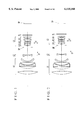•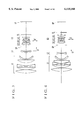•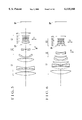•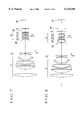•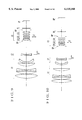•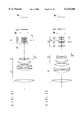•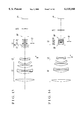•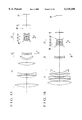••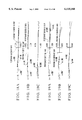•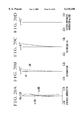•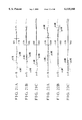•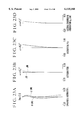•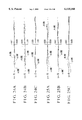•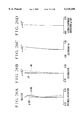•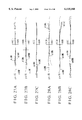•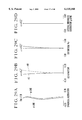•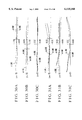•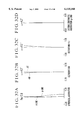•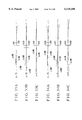•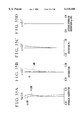•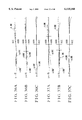•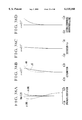•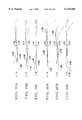••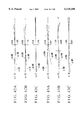•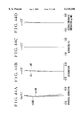•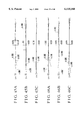•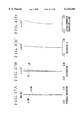•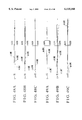••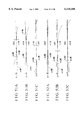•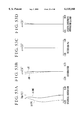•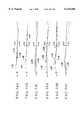•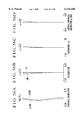•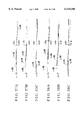•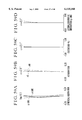•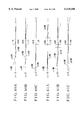•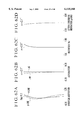•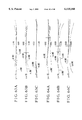•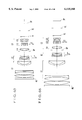•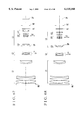•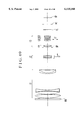•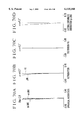•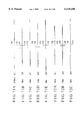•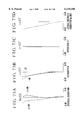•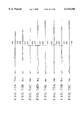•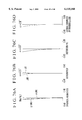•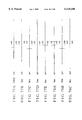•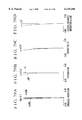•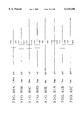•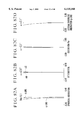•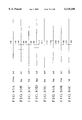•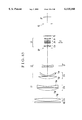•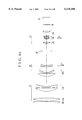•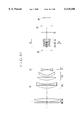•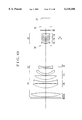•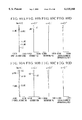•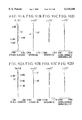•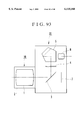## Classifications

• GPHYSICS
• G02OPTICS
• G02BOPTICAL ELEMENTS, SYSTEMS, OR APPARATUS
• G02B15/00Optical objectives with means for varying the magnification
• G02B15/14Optical objectives with means for varying the magnification by axial movement of one or more lenses or groups of lenses relative to the image plane for continuously varying the equivalent focal length of the objective
• G02B15/143Optical objectives with means for varying the magnification by axial movement of one or more lenses or groups of lenses relative to the image plane for continuously varying the equivalent focal length of the objective having three groups only
• G02B15/1431Optical objectives with means for varying the magnification by axial movement of one or more lenses or groups of lenses relative to the image plane for continuously varying the equivalent focal length of the objective having three groups only the first group being positive
• G02B15/143105Optical objectives with means for varying the magnification by axial movement of one or more lenses or groups of lenses relative to the image plane for continuously varying the equivalent focal length of the objective having three groups only the first group being positive arranged +-+
• GPHYSICS
• G02OPTICS
• G02BOPTICAL ELEMENTS, SYSTEMS, OR APPARATUS
• G02B15/00Optical objectives with means for varying the magnification
• G02B15/14Optical objectives with means for varying the magnification by axial movement of one or more lenses or groups of lenses relative to the image plane for continuously varying the equivalent focal length of the objective
• G02B15/16Optical objectives with means for varying the magnification by axial movement of one or more lenses or groups of lenses relative to the image plane for continuously varying the equivalent focal length of the objective with interdependent non-linearly related movements between one lens or lens group, and another lens or lens group
• G02B15/163Optical objectives with means for varying the magnification by axial movement of one or more lenses or groups of lenses relative to the image plane for continuously varying the equivalent focal length of the objective with interdependent non-linearly related movements between one lens or lens group, and another lens or lens group having a first movable lens or lens group and a second movable lens or lens group, both in front of a fixed lens or lens group
• G02B15/167Optical objectives with means for varying the magnification by axial movement of one or more lenses or groups of lenses relative to the image plane for continuously varying the equivalent focal length of the objective with interdependent non-linearly related movements between one lens or lens group, and another lens or lens group having a first movable lens or lens group and a second movable lens or lens group, both in front of a fixed lens or lens group having an additional fixed front lens or group of lenses
• G02B15/173Optical objectives with means for varying the magnification by axial movement of one or more lenses or groups of lenses relative to the image plane for continuously varying the equivalent focal length of the objective with interdependent non-linearly related movements between one lens or lens group, and another lens or lens group having a first movable lens or lens group and a second movable lens or lens group, both in front of a fixed lens or lens group having an additional fixed front lens or group of lenses arranged +-+

## Abstract

An optical system includes, in order from an object side, a first lens unit of positive refractive power, a second lens unit of negative refractive power, the second lens unit being movable along an optical axis to effect focusing, and a third lens unit of positive refractive power, the third lens unit including, in order from the object side, a first lens subunit of positive refractive power, a second lens subunit of negative refractive power and a third lens subunit of positive refractive power, wherein the second lens subunit is movable in directions perpendicular to the optical axis to displace an image formed by the optical system.

## Description

BACKGROUND OF THE INVENTION

1. Field of the Invention

The present invention relates to optical systems of the inner focus type suited to cameras for film, video cameras, video still cameras or the like.

2. Description of Related Art

It has been known to provide a lens type suited to the optical systems of long focal lengths, comprising, in order from an object side, a first lens unit of positive refractive power and a second lens unit of negative refractive power, or the so-called "telephoto" type. This lens type is advantageous at removing, in particular, spherical aberration and securing a fast F-number.

In, for example, Japanese Laid-Open Patent Applications No. Hei 5-27163, No. Hei 5-27164, No. Hei 6-242371, No. Hei 9-159911 and No. Hei 9-203859, the use of the telephoto type in optical systems has been proposed.

For many photographic lenses (optical systems,) focusing is performed by moving either the entirety of the complete lens, or one of the constituent lenses. In the case of long-focal-length lenses or telephoto lenses, because of their having bodies of large size and heavy weight, the former focusing method is hardly employed owing to the difficult problem of making up the operating mechanism.

For this reason, most of the telephoto lenses have the focusing provision made in one of the lens units. Of the lens units other than the front lens unit, a one in the central section is favorable for focusing purposes, because its size is relatively small and, moreover, because it is far light in weight. The use of this inner focus type has been proposed for various lenses.

For example, Japanese Laid-Open Patent Application No. Sho 55-147606 (U.S. Pat. No. 4,348,084) discloses a telephoto lens of the inner focus type having a focal length of 300 mm with an F-number of 2.8, and each of Japanese Laid-Open Patent Applications No. Sho 59-65820 and No. Sho 59-65821 discloses another telephoto lens of the inner focus type having a focal length of 135 mm with an F-number of 2.8.

The telephoto lenses of the inner focus type proposed in the above applications each comprise, in order from an object side, a first lens unit of positive refractive power, a second lens unit of negative refractive power and a third lens unit of positive refractive power, totaling three lens units, wherein the second lens unit is made axially movable for focusing.

Meanwhile, in shooting with the long-focal-length photographic system (optical system), it is generally difficult to suppress the influence of the vibrations of the photographic system. When the photographic system tilts due to the vibrations, the image on the focal plane changes its position out of alignment with a sight line, depending on the tilt angle and the focal length of the photographic system. Therefore, the photographic apparatus for taking still pictures suffers a problem of preventing the image quality from deteriorating by decreasing the exposure time to much short values. The motion-picture taking apparatus, too, encounters a difficult problem of keeping the composition at a desired setting. In such photographic situations, therefore, it becomes necessary to compensate for the tilting of the photographic system due to the vibrations so that the image on the focal plane remains stationary in alignment with the line of sight, that is, the so-called "shake" of the image does not occur.

There have been many methods of compensating for the shake of the image on the focal plane. For example, in the video cameras, the image pickup element is given a larger effective area than the necessary image frame and the image shake is electrically compensated for. Such an electric compensation method is widely employed in the video cameras.

In Japanese Laid-Open Patent Application No. Sho 61-223819, there is disclosed a photographic system in which a refractive-type variable angle prism is disposed at the frontmost position thereof. When the photographic system vibrates, the vertical angle of the variable angle prism is changed correspondingly with the vibration of the photographic system, so that an image is deflected so as to stabilize the image.

Another method of compensating for the shake of the image by moving a certain lens unit (movable lens unit) of an optical system in directions perpendicular to the optical axis was proposed in, for example, Japanese Laid-Open Patent Applications No. Sho 50-80147, No. Sho 61-223819, No. Hei 7-270724 (U.S. Pat. No. 5,646,779) and No. Hei 8-201691.

With such an optical system, if the relative aperture is increased (or if the F-number is made faster), the front lens unit becomes larger accordingly. As is well known, the F-number of the optical system is defined by

`Fno=f/D`

where Fno: the F-number,

f: the focal length of the optical system, and

D: the diameter of an effective light beam coming from an object point at infinity on the optical axis.

Accordingly, the large-relative-aperture telephoto lens (whose focal length is long) gets a large diameter for the equivalent F-number in direct proportion to the focal length. As the diameter of the front lens unit increases, the weight of the optical system increases rapidly. So, this tendency has been seen as problematic. Particularly for the photographic camera using 35 mm film, in the case of exceeding f=400 mm (semi-angle of field ω=3.1°), or in the case of the so-called "super" telephoto lens, this problem is very serious. In the locations where the tripod cannot be used, the hand-held shooting gives the photographer a heavy burden, becoming a cause of the image shake and an obstacle to the long-time shooting.

The method of compensating for the shake of the image by decentering a certain movable lens unit of the optical system so as to displace the image position is amenable to the techniques of minimizing the size of the apparatus by selecting a suitable one of the lens units for the movable lens unit.

However, the use of this method leads to taking into account two prerequisites, one of which is to properly select or arrange a lens unit of small size and light weight so that a lesser decentering movement produces a greater effect of displacing the image position, and the other of which is to prevent the image quality from deteriorating as far as possible, as that lens unit, when being decentered, produces aberrations. In general, it is very difficult to balance these conditions.

Meanwhile, the inner focus type, because of its using a lens unit of small size and light weight as the focusing lens, has merits that the management is easy, that a fast speed operation becomes possible, and that the holding is easy to keep, because the center of gravity of the whole lens system little changes between when focused on an infinitely distant object and when focused on an object at the minimum distance.

On the other hand, the use of the inner focus type in the telephoto lens of fast F-number increases the range of variation of aberrations with focusing. This variation of aberrations, because of being difficult to correct well, comes to a cause of lowering the optical performance.

BRIEF SUMMARY OF THE INVENTION

The present invention is concerned with the image stabilizing function in the optical system which makes one lens unit movable to decenter in directions perpendicular to an optical axis. With this regard, an object of the invention is to provide an optical system which can manifest the image stabilizing function, while still permitting the decentering aberrations to be as far suppressed as possible.

The present invention is concerned also with the inner focus type. Another object of the invention is, therefore, to provide an optical system which suppresses the variation with focusing of aberrations as far as possible over a wide range of object distances.

To attain the former object, in accordance with an aspect of the invention, there is provided an optical system, which comprises, in order from an object side, a first lens unit of positive refractive power, a second lens unit of negative refractive power, the second lens unit being movable along an optical axis to effect focusing, and a third lens unit of positive refractive power, the third lens unit including, in order from the object side, a first lens subunit of positive refractive power, a second lens subunit of negative refractive power and a third lens subunit of positive refractive power, wherein the second lens subunit is movable in directions perpendicular to the optical axis to displace an image formed by the optical system.

To attain the latter object, in accordance with another aspect of the invention, there is provided an optical system, which comprises, in order from an object side, a first lens unit of positive refractive power, the first lens unit consisting of, with boundaries formed by the widest and the second-widest spaces thereof, in order from the object side, a front lens subunit of positive refractive power, a middle lens subunit of negative refractive power and a rear lens subunit of positive refractive power, and a second lens unit of negative refractive power, the second lens unit being movable along an optical axis to effect focusing, wherein, letting a focal length of the entire optical system be denoted by f, a focal length of the front lens subunit be denoted by f1a, and a space between the front lens subunit and the rear lens subunit be denoted by D1ab, the following conditions are satisfied:

`0.4<f1a/f<1.1`

`0.035<D1ab/f<0.15.`

These and further objects and aspects of the invention will become apparent from the following detailed description of preferred embodiments thereof taken in conjunction with the accompanying drawings.

BRIEF DESCRIPTION OF THE SEVERAL VIEWS OF THE DRAWING

FIG. 1 is a longitudinal section view of a numerical example 1 of the lens.

FIG. 2 is a longitudinal section view of a numerical example 2 of the lens.

FIG. 3 is a longitudinal section view of a numerical example 3 of the lens.

FIG. 4 is a longitudinal section view of a numerical example 4 of the lens.

FIG. 5 is a longitudinal section view of a numerical example 5 of the lens.

FIG. 6 is a longitudinal section view of a numerical example 6 of the lens.

FIG. 7 is a longitudinal section view of a numerical example 7 of the lens.

FIG. 8 is a longitudinal section view of a numerical example 8 of the lens.

FIG. 9 is a longitudinal section view of a numerical example 9 of the lens.

FIG. 10 is a longitudinal section view of a numerical example 10 of the lens.

FIG. 11 is a longitudinal section view of a numerical example 11 of the lens.

FIG. 12 is a longitudinal section view of a numerical example 12 of the lens.

FIG. 13 is a longitudinal section view of a numerical example 13 of the lens.

FIG. 14 is a longitudinal section view of a numerical example 14 of the lens.

FIG. 15 is a longitudinal section view of a numerical example 15 of the lens.

FIG. 16 is a longitudinal section view of a numerical example 16 of the lens.

FIGS. 17A to 17D are graphic representations of the longitudinal aberrations of the numerical example 1 in the normal state (with the image position unchanged).

FIGS. 18A to 18C are graphic representations of the lateral aberrations of the numerical example 1 in the normal state (with the image position unchanged).

FIGS. 19A to 19C are graphic representations of the lateral aberrations of the numerical example 1 when the image of an infinitely distant object has displaced to a corresponding position to 0.3° in image angle.

FIGS. 20A to 20D are graphic representations of the longitudinal aberrations of the numerical example 2 in the normal state (with the image position unchanged).

FIGS. 21A to 21C are graphic representations of the lateral aberrations of the numerical example 2 in the normal state (with the image position unchanged).

FIGS. 22A to 22C are graphic representations of the lateral aberrations of the numerical example 2 when the image of an infinitely distant object has displaced to a corresponding position to 0.3° in image angle.

FIGS. 23A to 23D are graphic representations of the longitudinal aberrations of the numerical example 3 in the normal state (with the image position unchanged).

FIGS. 24A to 24C are graphic representations of the lateral aberrations of the numerical example 3 in the normal state (with the image position unchanged).

FIGS. 25A to 25C are graphic representations of the lateral aberrations of the numerical example 3 when the image of an infinitely distant object has displaced to a corresponding position to 0.3° in image angle.

FIGS. 26A to 26D are graphic representations of the longitudinal aberrations of the numerical example 4 in the normal state (with the image position unchanged).

FIGS. 27A to 27C are graphic representations of the lateral aberrations of the numerical example 4 in the normal state (with the image position unchanged).

FIGS. 28A to 28C are graphic representations of the lateral aberrations of the numerical example 4 when the image of an infinitely distant object has displaced to a corresponding position to 0.3° in image angle.

FIGS. 29A to 29D are graphic representations of the longitudinal aberrations of the numerical example 5 in the normal state (with the image position unchanged).

FIGS. 30A to 30C are graphic representations of the lateral aberrations of the numerical example 5 in the normal state (with the image position unchanged).

FIGS. 31A to 31C are graphic representations of the lateral aberrations of the numerical example 5 when the image of an infinitely distant object has displaced to a corresponding position to 0.3° in image angle.

FIGS. 32A to 32D are graphic representations of the longitudinal aberrations of the numerical example 6 in the normal state (with the image position unchanged).

FIGS. 33A to 33C are graphic representations of the lateral aberrations of the numerical example 6 in the normal state (with the image position unchanged).

FIGS. 34A to 34C are graphic representations of the lateral aberrations of the numerical example 6 when the image of an infinitely distant object has displaced to a corresponding position to 0.3° in image angle.

FIGS. 35A to 35D are graphic representations of the longitudinal aberrations of the numerical example 7 in the normal state (with the image position unchanged).

FIGS. 36A to 36C are graphic representations of the lateral aberrations of the numerical example 7 in the normal state (with the image position unchanged).

FIGS. 37A to 37C are graphic representations of the lateral aberrations of the numerical example 7 when the image of an infinitely distant object has displaced to a corresponding position to 0.3° in image angle.

FIGS. 38A to 38D are graphic representations of the longitudinal aberrations of the numerical example 8 in the normal state (with the image position unchanged).

FIGS. 39A to 39C are graphic representations of the lateral aberrations of the numerical example 8 in the normal state (with the image position unchanged).

FIGS. 40A to 40C are graphic representations of the lateral aberrations of the numerical example 8 when the image of an infinitely distant object has displaced to a corresponding position to 0.3° in image angle.

FIGS. 41A to 41D are graphic representations of the longitudinal aberrations of the numerical example 9 in the normal state (with the image position unchanged).

FIGS. 42A to 42C are graphic representations of the lateral aberrations of the numerical example 9 in the normal state (with the image position unchanged).

FIGS. 43A to 43C are graphic representations of the lateral aberrations of the numerical example 9 when the image of an infinitely distant object has displaced to a corresponding position to 0.3° in image angle.

FIGS. 44A to 44D are graphic representations of the longitudinal aberrations of the numerical example 10 in the normal state (with the image position unchanged).

FIGS. 45A to 45C are graphic representations of the lateral aberrations of the numerical example 10 in the normal state (with the image position unchanged).

FIGS. 46A to 46C are graphic representations of the lateral aberrations of the numerical example 10 when the image of an infinitely distant object has displaced to a corresponding position to 0.3° in image angle.

FIGS. 47A to 47D are graphic representations of the longitudinal aberrations of the numerical example 11 in the normal state (with the image position unchanged).

FIGS. 48A to 48C are graphic representations of the lateral aberrations of the numerical example 11 in the normal state (with the image position unchanged).

FIGS. 49A to 49C are graphic representations of the lateral aberrations of the numerical example 11 when the image of an infinitely distant object has displaced to a corresponding position to 0.3° in image angle.

FIGS. 50A to 50D are graphic representations of the longitudinal aberrations of the numerical example 12 in the normal state (with the image position unchanged).

FIGS. 51A to 51C are graphic representations of the lateral aberrations of the numerical example 12 in the normal state (with the image position unchanged).

FIGS. 52A to 52C are graphic representations of the lateral aberrations of the numerical example 12 when the image of an infinitely distant object has displaced to a corresponding position to 0.3° in image angle.

FIGS. 53A to 53D are graphic representations of the longitudinal aberrations of the numerical example 13 in the normal state (with the image position unchanged).

FIGS. 54A to 54C are graphic representations of the lateral aberrations of the numerical example 13 in the normal state (with the image position unchanged).

FIGS. 55A to 55C are graphic representations of the lateral aberrations of the numerical example 13 when the image of an infinitely distant object has displaced to a corresponding position to 0.3° in image angle.

FIGS. 56A to 56D are graphic representations of the longitudinal aberrations of the numerical example 14 in the normal state (with the image position unchanged).

FIGS. 57A to 57C are graphic representations of the lateral aberrations of the numerical example 14 in the normal state (with the image position unchanged).

FIGS. 58A to 58C are graphic representations of the lateral aberrations of the numerical example 14 when the image of an infinitely distant object has displaced to a corresponding position to 0.3° in image angle.

FIGS. 59A to 59D are graphic representations of the longitudinal aberrations of the numerical example 15 in the normal state (with the image position unchanged).

FIGS. 60A to 60C are graphic representations of the lateral aberrations of the numerical example 15 in the normal state (with the image position unchanged).

FIGS. 61A to 61C are graphic representations of the lateral aberrations of the numerical example 15 when the image of an infinitely distant object has displaced to a corresponding position to 0.3° in image angle.

FIGS. 62A to 62D are graphic representations of the longitudinal aberrations of the numerical example 16 in the normal state (with the image position unchanged).

FIGS. 63A to 63C are graphic representations of the lateral aberrations of the numerical example 16 in the normal state (with the image position unchanged).

FIGS. 64A to 64C are graphic representations of the lateral aberrations of the numerical example 16 when the image of an infinitely distant object has displaced to a corresponding position to 0.3° in image angle.

FIG. 65 is a longitudinal section view of a numerical example 17 of the lens.

FIG. 66 is a longitudinal section view of a numerical example 18 of the lens.

FIG. 67 is a longitudinal section view of a numerical example 19 of the lens.

FIG. 68 is a longitudinal section view of a numerical example 20 of the lens.

FIG. 69 is a longitudinal section view of a numerical example 21 of the lens.

FIGS. 70A to 70D are graphic representations of the longitudinal aberrations of the numerical example 17 in the normal state (with the image position unchanged).

FIGS. 71A to 71E are graphic representations of the lateral aberrations of the numerical example 17 in the normal state (with the image position unchanged).

FIGS. 72A to 72C are graphic representations of the lateral aberrations of the numerical example 17 when the image of an infinitely distant object has displaced to a corresponding position to 0.5° in image angle.

FIGS. 73A to 73D are graphic representations of the longitudinal aberrations of the numerical example 18 in the normal state (with the image position unchanged).

FIGS. 74A to 74E are graphic representations of the lateral aberrations of the numerical example 18 in the normal state (with the image position unchanged).

FIGS. 75A to 75C are graphic representations of the lateral aberrations of the numerical example 18 when the image of an infinitely distant object has displaced to a corresponding position to 0.5° in image angle.

FIGS. 76A to 76D are graphic representations of the longitudinal aberrations of the numerical example 19 in the normal state (with the image position unchanged).

FIGS. 77A to 77E are graphic representations of the lateral aberrations of the numerical example 19 in the normal state (with the image position unchanged).

FIGS. 78A to 78C are graphic representations of the lateral aberrations of the numerical example 19 when the image of an infinitely distant object has displaced to a corresponding position to 0.5° in image angle.

FIGS. 79A to 79D are graphic representations of the longitudinal aberrations of the numerical example 20 in the normal state (with the image position unchanged).

FIGS. 80A to 80E are graphic representations of the lateral aberrations of the numerical example 20 in the normal state (with the image position unchanged).

FIGS. 81A to 81C are graphic representations of the lateral aberrations of the numerical example 20 when the image of an infinitely distant object has displaced to a corresponding position to 0.5° in image angle.

FIGS. 82A to 82D are graphic representations of the longitudinal aberrations of the numerical example 21 in the normal state (with the image position unchanged).

FIGS. 83A to 83E are graphic representations of the lateral aberrations of the numerical example 21 in the normal state (with the image position unchanged).

FIGS. 84A to 84C are graphic representations of the lateral aberrations of the numerical example 21 when the image of an infinitely distant object has displaced to a corresponding position to 0.5° in image angle.

FIG. 85 is a longitudinal section view of a numerical example 22 of the lens.

FIG. 86 is a longitudinal section view of a numerical example 23 of the lens.

FIG. 87 is a longitudinal section view of a numerical example 24 of the lens.

FIG. 88 is a longitudinal section view of a numerical example 25 of the lens.

FIGS. 89A to 89D are graphic representations of the various aberrations of the numerical example 22.

FIGS. 90A to 90D are graphic representations of the various aberrations of the numerical example 23.

FIGS. 91A to 91D are graphic representations of the various aberrations of the numerical example 24.

FIGS. 92A to 92D are graphic representations of the various aberrations of the numerical example 25.

FIG. 93 is a schematic view of the main parts of a single-lens reflex camera according to a fourth embodiment.

DETAILED DESCRIPTION OF THE INVENTION

Hereinafter, preferred embodiments of the invention will be described in detail with reference to the drawings.

(First Embodiment)

FIGS. 1 to 16 in lens block diagrams show sixteen numerical examples 1 to 16 of a first embodiment of the invention, respectively. Each optical system comprises, in order from an object side, a first lens unit L1 of positive refractive power, a second lens unit L2 of negative refractive power and a third lens unit L3 of positive refractive power.

The third lens unit L3 includes three lens subunits, i.e., a first lens subunit L31 of positive refractive power, a second lens subunit L32 of negative refractive power and a third lens subunit L33 of positive refractive power. SP stands for an aperture stop, FL stands for an optical filter, and IP stands for an image plane.

In the present embodiment, during focusing from an infinitely distant object to shorter object distances, the second lens unit L2 axially moves toward the image side as indicated by an arrow LF. Also, when the optical system vibrates, the shake of the image on the image plane IP is compensated for (or the image is stabilized) by moving the second lens subunit L32 in directions perpendicular to an optical axis, as shown by a double-headed arrow LT.

According to the present embodiment, the optical system has a feature that, as compared with the first lens unit L1, the second lens unit L2 is small in diameter and far lighter in weight and has a negative refractive power. This second lens unit L2 is selected to be used as the focusing lens. So, an electric motor of low torque and small size can be utilized in a drive device therefor. Another feature is that the overall refractive power of the first and second lens units L1 and L2 is made positive. As the second lens unit L2 produces a convergent light beam, the first lens subunit L31 further converges it. This makes it easier to minimize the diameter of the second lens subunit L32 (as the image displacement compensating unit). Still another feature is that the third lens subunit L33 of positive refractive power follows the second lens subunit L32. This allows the negative refractive power of the second lens subunit L32 to increase, while keeping the constant focal length of the entire optical system, thereby making it easier to translate a lesser decentering movement of the second lens subunit L32 to the displacement large enough of the image on the focal plane. (The relationship between the decentered amount and the image displacement is hereinafter called the "image displacement sensitivity".)

The present embodiment has set forth such features to stabilize the image well when the user uses the video camera or still camera with the telephoto lens held by his or her hands, or on an unsteady tripod or mono-pod.

In the present embodiment, not only the inner-focusing and the compensation for the image shake on the focal plane are carried out as such, but also proper rules of design for the optical parameters of each of the lens units are determined. Accordingly, the image shake is compensated for well in such a manner as to assure minimization of the bulk and size of the entire optical system. In addition, as the second lens subunit L32 moves in the directions perpendicular to the optical axis, lesser decentering aberrations, especially coma, astigmatism and field curvature, are produced and a good optical performance is maintained stable throughout the entire focusing range.

To facilitate the reduction of the produced amount of decentering aberrations and the maintenance of good stability of optical performance throughout the entire focusing range, it is preferred to satisfy at least one of the following features or conditions.

(a-1) The second lens subunit L32 has an air lens of bi-convex form, and the following condition is satisfied:

`0.6<fair/f32<1.4                                           (1)`

where "fair" is the focal length of the air lens, and f32 is the focal length of the second lens subunit L32. Incidentally, the focal length of an air lens is determined by the refractive indices of the materials disposed before and behind the air lens.

The inequalities of condition (1) are provided for creating the air lens of appropriate shape in the interior of the second lens subunit L32 to obtain a good image quality in either of the normal state (with the image not displaced) and the image-stabilized state. When the numerical range of the condition (1) is exceeded, it becomes difficult to take a balance of the image quality between the normal state and the image-displayed state.

(a-2) The second lens subunit L32 has an air lens of bi-convex form, and the following condition is satisfied: ##EQU1## where Rair1 and Rair2 are radii of curvature of lens surfaces on the object side and the image side of the air lens, respectively.

The inequalities of condition (2) give an appropriate range for the radii of curvature of lens surfaces on the object side and the image side of the air lens so as to further improve the optical performance. When the range of the condition (2) is exceeded, similarly to the condition (1), it becomes difficult to take a balance of the image quality between the normal state and the image-displaced state.

(a-3) Letting the focal length of the i-th lens unit be denoted by fi, and the focal lengths of the first lens subunit L31, the second lens subunit L32 and the third lens subunit L33 be denoted by f31, f32 and f33, respectively, the following conditions are satisfied:

`0.3<f1/f<0.75                                              (3)`

`0.2<|f2/f|<0.7                           (4)`

`0.1<f/f3<1.5                                               (5)`

`0.12<f31/f<0.5                                             (6)`

`0.05<|f32/f|<0.15                        (7)`

`0.08<f33/f<0.25                                            (8)`

It is to be noted that the conditions (3) to (8) are not necessary to satisfy at once; so they may be selectively satisfied. Even in this case, their results to be described later are obtained individually.

The technical significance of each of the conditions (3) to (8) is next described below.

The inequalities of condition (3) give a proper range for the refractive power of the positive first lens unit L1. When the upper limit of the condition (3) is exceeded, as this means that the refractive power of the first lens unit L1 is too weak, the telephoto ability weakens, causing the total length of the entire optical system to increase objectionably. The converging action also becomes too weak. Therefore, a bulky light bundle enters the second lens unit L2. To admit of this, the diameter of the second lens unit L2 has to be increased greatly. Also, to keep the constant angle of field, the refractive power of the second lens unit L2 has to be weakened. Therefore, the total focusing movement increases greatly. When the lower limit is exceeded, as this means that the positive refractive power is too strong, spherical aberrations of high orders are produced, which are hardly corrected by the other lens units.

The inequalities of condition (4) give a proper range for the refractive power of the focusing, or negative second, lens unit L2. When the upper limit of the condition (4) is exceeded, the focusing movement from infinity to a certain finite distance increases to hinder minimization of the size of the lens system. Also, the second lens unit L2 of negative refractive power can no longer correct the various aberrations, especially spherical aberration, produced by the first lens unit L1 of positive refractive power. When the lower limit is exceeded, the spherical aberration is over-corrected by the second lens unit L2 of negative refractive power. As a result, it becomes difficult to retain the good correction of the image aberrations.

The inequalities of condition (5) give a proper range for the refractive power of the third lens unit L3 of positive refractive power. When the upper limit of the condition (5) is exceeded, too long a back focal distance results. Therefore, the size of the entire optical system is hardly minimized and, moreover, the positive distortion produced by the first lens unit becomes difficult to correct.

When the lower limit is exceeded, the number of lens elements in the third lens unit has to be increased so as to correct spherical aberration, coma flare or the like. As a result, the size of the entire optical system is hardly minimized and, moreover, the light transmittance of the optical system worsens. So, these violations are objectionable.

The inequalities of conditions (6) to (8) give proper ranges for the refractive powers of the lens subunits in the third lens unit, and have an aim that, as the second lens subunit L32 axially moves in the directions perpendicular to the optical axis to stabilize the image, a high sensitivity of image displacement is obtained while still securing the good imaging performance. When the numerical ranges of these conditions (6) to (8) are exceeded, it becomes difficult to keep the balance therebetween.

(a-4) The second lens unit L2 has at least one positive lens and at least one negative lens, and, letting the mean Abbe numbers of materials of the positive lens and the negative lens be denoted by νp and νn, respectively, the following condition is satisfied:

`7.5<νn-νp<30                                         (9)`

In the present embodiment, the second lens unit L2 must be decreased in weight to decrease the torque of the focusing driver and the variation with focusing of aberrations must be reduced. To these purposes, it is preferred to construct the second lens unit L2 with a positive lens having a stronger convex surface facing the image side than the object side and a negative lens having a stronger concave surface facing the image side than the object side, as arranged in this order from the object side. The inequalities of condition (9) give a proper range for the difference between the Abbe numbers of materials of the positive and negative lenses and have an aim to correct well the variation with focusing of chromatic aberrations.

It is to be noted that, in a case where the second lens unit L2 has its refractive power made strong in order to reduce its total focusing movement, two or more negative lenses may be used.

(a-5) The second lens subunit L32 consists of, in order from the object side, a positive lens of bi-convex form and two negative lenses of bi-concave form. With this arrangement, when stabilizing the image, the good optical performance is maintained stable.

(a-6) The second lens subunit L32 consists of, in order from the object side, a negative lens of meniscus form convex toward the object side, a positive lens of meniscus form convex toward the object side and a negative lens of bi-concave form. With this arrangement, when stabilizing the image, the good optical performance is maintained stable.

(a-7) The second lens unit L2 consists of a positive lens and a negative lens. With this arrangement, the variation with focusing of chromatic aberrations is corrected well.

(a-8) The third lens subunit L33 has a plurality of positive lenses. To achieve a high sensitivity of image displacement, the third lens subunit L33 is better given a somewhat strong positive refractive power. Particularly for a large relative aperture lens system to achieve, it is preferred that the third lens subunit L33 has a plurality of positive lenses with an advantage of correcting, among others, spherical aberrations of higher orders. If the third lens subunit L33 further includes at least one positive cemented lens composed of negative and positive lenses, or at least one pair of positive and negative lenses with their confronting surfaces of nearly equal radii of curvature to each other, even more improved chromatic aberrations can be expected.

(a-9) In order to make it easier to correct the residual aberrations produced by the first and second lens units L1 and L2 by a lens unit on the image side, the first lens sub unit L31 is desired to have at least one positive lens and at least one negative lens. Also, the second lens subunit L32 is desired to have a plurality of negative lenses and at least one positive lens. With this arrangement, when stabilizing the image, the variation of chromatic aberrations can be reduced.

(a-10) The first lens unit L1 is better constructed with a plurality of positive lenses and at least one negative lens. To attain a high image quality, the first lens unit L1 is better constructed with, in order from the object side, a positive lens of bi-convex form, a positive lens whose front surface is strong convex, a bi-concave lens and a positive lens whose front surface is strong convex. More preferably, these lenses are followed by a negative lens of meniscus form convex toward the object side.

(a-11) To protect the exposed lens surface to the outside and prevent occurrence of a change of the position of the sharp focus owing to the expansion or contraction of the lens elements with change of the ambient temperature, use may be made of a flat glass plate or transparent member or a lens of weak refractive power on the object side of the lens system.

(a-12) An iris diaphragm (aperture stop SP) may take any position whatever in the lens system, provided that, when stopped down, the light beam which goes to the margin of the useful image circle is not vignetted. On consideration of the space efficiency of layout of the drive mechanism for the focusing lens unit, the moving mechanism for the image stabilizing subunit and the mother board of electric circuitry, however, the iris diaphragm is better positioned in the air space between the second lens unit L2 and the third lens unit L3, or in the interior of the third lens unit L3.

(a-13) The optical filter FL is positioned in the space between the rearmost lens surface and the image plane, as desired because of assuring minimization of the diameter of the filter and from the point of view of the space efficiency.

(a-14) A fixed stop HP is better positioned in the air space between the rearmost lens surface and the image plane. With this arrangement, when stabilizing the image, the asymmetry of corner illuminations can be reduced.

(a-15) It is more preferred that the numerical ranges of the above-described conditions (1) to (9) are made narrower as follows:

`0.7<fair/f32<1.2                                           (1a) ##EQU2##`

`0.4<f1/f<0.7                                               (3a)`

`0.25<|f2/f|<0.6                          (4a)`

`0.1<f/f3<1.2                                               (5a)`

`0.15<f31/f<0.45                                            (6a)`

`0.06<|f32/f|<0.14                        (7a)`

`0.11<f33/f<0.23                                            (8a)`

`8.0<νn×νp<27                                   (9a)`

Next, sixteen numerical examples 1 to 16 of the first embodiment are shown. In the numerical data for the examples 1 to 16, Ri is the radius of curvature of the i-th lens surface, when counted from the object side, Di is the i-th axial lens thickness or air separation, when counted from the object side, and Ni and νi are respectively the refractive index and Abbe number of the material of the i-th lens element, when counted from the object side. In the numerical examples 1 to 7 and 9 to 16, the last two surfaces define a glass block such as phase plate or filter. In the numerical example 8, the second and third surfaces from the last define a glass block. The values of the factors in the above-described conditions (1) to (9) for the numerical examples 1 to 16 are listed in Table-1.

`______________________________________Numerical Example 1:______________________________________f = 293.58 Fno = 2.9 2ω = 8.4°R1 = 130.154      D1 = 16.56 N1 = l.496999                              ν1 = 81.5R2 = -352.589      D2 = 14.11R3 = 96.004      D3 = 11.02 N2 = 1.496999                              ν2 = 81.5R4 = 793.095      D4 = 4.07R5 = -311.697      D5 = 4.00  N3 = 1.785896                              ν3 = 44.2R6 = 127.604      D6 = 0.15R7 = 79.467      D7 = 13.88 N4 = 1.433870                              ν4 = 95.1R8 = -483.755      D8 = Q.17R9 = 51.083      D9 = 5.90  N5 = 1.487490                              ν5 = 70.2R10 = 40.134      D10 = 26.71R11 = 1686.885      D11 = 4.61 N6 = 1.805181                              ν6 = 25.4R12 = -107.660      D12 = 2.20 N7 = 1.834807                              ν7 = 42.7R13 = 94.265      D13 = 40.40R14 = Stop D14 = 8.51R15 = 79.490      D15 = 1.80 N8 = 1.846658                              ν8 = 23.9R16 = 35.239      D16 = 7.20 N9 = 1.719995                              ν9 = 50.2R17 = -238.114      D17 = 0.95R18 = 129.720      D18 = 4.25 N10 = 1.846658                              ν10 = 23.9R19 = -97.425      D19 = 1.65 N11 = 1.603112                              ν11 = 60.6R20 = 36.658      D20 = 5.52R21 = -76.051      D21 = 1.60 N12 = 1.772499                              ν12 = 49.6R22 = 66.247      D22 = 2.82R23 = 78.646      D23 = 9.30 N13 = 1.719995                              ν13 = 50.2R24 = -39.586      D24 = 1.80 N14 = 1.834000                              ν14 = 37.2R25 = -155.699      D25 = 4.00R26 = 81.751      D26 = 5.50 N15 = 1.696797                              ν15 = 55.5R27 = ∞      D27 = 8.00R28 = ∞      D28 = 2.00 N16 = 1.516330                              ν16 = 64.1R29 = ∞      D29 = 0.00______________________________________Numerical Example 2______________________________________f = 294.17 Fno = 2.9 2ω = 8.4°R1 = 127.473      D1 = 17.00 N1 = 1.433870                              ν1 = 95.1R2 = -307.517      D2 = 10.73R3 = 99.685      D3 = 11.30 N2 = 1.496999                              ν2 = 81.6R4 = 643.990      D4 = 5.85R5 = -304.401      D5 = 4.50  N3 = 1.772499                              ν3 = 49.6R6 = 121.012      D6 = 0.15R7 = 83.253      D7 = 14.00 N4 = 1.496999                              ν4 = 81.6R8 = -619.135      D8 = 0.15R9 = 52.533      D9 = 5.80  N5 = 1.603420                              ν5 = 38.0R10 = 42.498      D10 = 32.70R11 = 1185.504      D11 = 3.76 N6 = 1.846659                              ν6 = 23.8R12 = -164.476      D12 = 0.00R13 = -164.476      D13 = 2.20 N7 = 1.834807                              ν7 = 42.7R14 = 98.692      D14 = 47.87R15 = Stop D15 = 6.05R16 = 70.883      D16 = 1.80 N8 = 1.846659                              ν8 = 23.8R17 = 29.520      D17 = 8.40 N9 = 1.785896                              ν9 = 44.2R18 = -767.243      D18 = 0.95R19 = 78.009      D19 = 4.87 N10 = 1.846659                              ν10 = 23.8R20 = -87.548      D20 = 1.65 N11 = 1.696797                              ν11 = 55.5R21 = 33.742      D21 = 4.84R22 = -85.087      D22 = 1.60 N12 = 1.834807                              ν12 = 42.7R23 = 59.332      D23 = 2.16R24 = 97.295      D24 = 8.00 N13 = 1.749497                              ν13 = 35.3R25 = -38.518      D25 = 1.80 N14 = 1.805181                              ν14 = 25.4R26 = -182.752      D26 = 0.15R27 = 53.888      D27 = 5.40 N15 = 1.516330                              ν15 = 64.2R28 = -1394.217      D28 = 10.00R29 = -1394.217      D29 = 2.00 N16 = 1.516330                              ν16 = 64.2R30 = -1394.217      D30 = 0.00______________________________________Numerical Example 3:______________________________________f = 294.60 Fno = 2.9 2ω = 8.4°R1 = 155.346      D1 = 17.00 N1 = 1.496999                              ν1 = 81.6R2 = -255.011      D2 = 0.15R3 = 105.911      D3 = 12.00 N2 = 1.438750                              ν2 = 95.0R4 = 1180.529      D4 = 4.36R5 = -309.541      D5 = 4.50  N3 = 1.772499                              ν3 = 49.6R6 = 140.895      D6 = 14.92R7 = 80.148      D7 = 15.00 N4 = 1.496999                              ν4 = 81.6R8 = 13727.840      D8 = 0.15R9 = 53.615      D9 = 5.00  N5 = 1.581439                              ν5 = 40.8R10 = 43.375      D10 = 34.00R11 = 167.036      D11 = 3.99 N6 = 1.846659                              ν6 = 23.8R12 = -683.959      D12 = 0.58R13 = -425.247      D13 = 2.20 N7 = 1.882997                              ν7 = 40.8R14 = 80.847      D14 = 22.54R15 = Stop D15 = 7.64R16 = 151.995      D16 = 1.80 N8 = 1.846658                              ν8 = 23.9R17 = 39.448      D17 = 8.70 N9 = 1.816000                              ν9 = 46.6R18 = -229.473      D18 = 1.00R19 = 69.553      D19 = 5.00 N10 = 1.846658                              ν10 = 23.9R20 = -140.993      D20 = 1.70 N11 = 1.754998                              ν11 = 52.3R21 = 34.675      D21 = 5.52R22 = -109.578      D22 = 1.65 N12 = 1.834807                              ν12 = 42.7R23 = 80.898      D23 = 2.50R24 = 118.731      D24 = 4.70 N13 = 1.725999                              ν13 = 53.6R25 = -241.783      D25 = 2.64R26 = 52.272      D26 = 6.00 N14 = 1.487490                              ν14 = 70.2R27 = 323.032      D27 = 10.00R28 = ∞      D28 = 2.00 N15 = 1.516330                              ν15 = 64.2R29 = ∞      D29 = 0.00______________________________________Numerical Example 4:______________________________________f = 293.97 Fno = 2.9 2ω = 8.4°R1 = 137.107      D1 = 17.30 N1 = 1.496999                              ν1 = 81.6R2 = -283.319      D2 = 2.31R3 = 89.821      D3 = 13.20 N2 = 1.496999                              ν2 = 81.6R4 = 711.950      D4 = 4.37R5 = -332.080      D5 = 4.00  N3 = 1.756998                              ν3 = 47.8R8 = 117.445      D6 = 0.15R7 = 76.079      D7 = 16.50 N4 = 1.433870                              ν4 = 95.1R8 = -479.886      D8 = 0.15R9 = 44.980      D9 = 5.90  N5 = 1.625882                              ν5 = 35.7R10 = 36.558      D10 = 24.40R11 = 3005.033      D11 = 4.29 N6 = 1.846659                              ν6 = 23.8R12 = -117.913      D12 = 0.00R13 = -117.913      D13 = 2.20 N7 = 1.834807                              ν7 = 42.7R14 = 75.547      D14 = 40.16R15 = Stop D15 = 8.91R16 = 63.764      D16 = 1.80 N8 = 1.846658                              ν8 = 23.9R17 = 31.830      D17 = 8.40 N9 = 1.696797                              ν9 = 55.5R18 = -301.122      D18 = 0.95R19 = 79.604      D19 = 4.71 N10 = 1.846659                              ν10 = 23.8R20 = -117.900      D20 = 1.70 N11 = 1.651597                              ν11 = 58.5R21 = 30.513      D21 = 5.93R22 = -69.281      D22 = 1.65 N12 = 1.834807                              ν12 = 42.7R23 = 79.237      D23 = 1.81R24 = 87.567      D24 = 6.00 N13 = 1.518229                              ν13 = 59.0R25 = -131.515      D25 = 4.34R26 = 63.796      D26 = 6.50 N14 = 1.563839                              ν14 = 60.7R27 = -274.113      D27 = 10.00R28 = ∞      D28 = 2.00 N15 = 1.516330                              ν15 = 64.2R29 = ∞      D29 = 0.00______________________________________Numerical Example 5:______________________________________f = 294.60 Fno = 2.9 2ω = 8.4°R1 = 160.901      D1 = 17.50 N1 = 1.487490                              ν1 = 70.2R2 = -236.537      D2 = 1.47R3 = 109.787      D3 = 13.00 N2 = 1.438750                              ν2 = 95.0R4 = -36510.670      D4 = 4.10R5 = -252.975      D5 = 4.50  N3 = 1.804000                              ν3 = 46.6R6 = 160.446      D6 = 29.63R7 = 91.963      D7 = 17.00 N4 = 1.438750                              ν4 = 95.0R8 = -303.068      D8 = 2.44R9 = 49.410      D9 = 5.50  N5 = 1.516800                              ν5 = 64.2R10 = 41.061      D10 = 34.00R11 = 258.070      D11 = 4.34 N6 = 1.846659                              ν6 = 23.8R12 = -148.644      D12 = 0.24R13 = -143.583      D13 = 2.20 N7 = 1.882997                              ν7 = 40.8R14 = 73.831      D14 = 22.64R15 = Stop D15 = 6.89R16 = 86.175      D16 = 1.80 N8 = 1.846658                              ν8 = 23.9R17 = 37.454      D17 = 8.70 N9 = 1.740999                              ν9 = 52.6R18 = -251.183      D18 = 1.00R19 = 72.319      D19 = 5.00 N10 = 1.846658                              ν10 = 23.9R20 = -265.500      D20 = 1.70 N11 = 1.677900                              ν11 = 55.3R21 = 32.565      D21 = 5.09R22 = -79.925      D22 = 1.65 N12 = 1.834807                              ν12 = 42.7R23 = 81.766      D23 = 2.00R24 = 95.485      D24 = 4.70 N13 = 1.589130                              ν13 = 61.2R25 = -358.724      D25 = 2.50R26 = 64.449      D26 = 6.00 N14 = 1.620410                              ν14 = 60.3R27 = -323.156      D27 = 10.00R28 = ∞      D28 = 2.00 N15 = 1.516330                              ν15 = 64.2R29 = ∞      D29 = 0.00______________________________________Numerical Example 6:______________________________________f = 294.59 Fno = 2.9 2ω = 8.4°R1 = 109.004      D1 = 20.00 N1 = 1.438750                              ν1 = 95.0R2 = -337.044      D2 = 7.68R3 = 120.763      D3 = 10.68 N2 = 1.496999                              ν2 = 81.6R4 = 1437.484      D4 = 3.40R5 = -357.888      D5 = 4.50  N3 = 1.834807                              ν3 = 42.7R6 = 162.770      D6 = 2.19R7 = 66.497      D7 = 12.00 N4 = 1.496999                              ν4 = 81.6R8 = 213.508      D8 = 8.57R9 = 78.563      D9 = 4.30  N5 = 1.783000                              ν5 = 36.1R10 = 46.466      D10 = 11.47R11 = 70.759      D11 = 6.00 N6 = 1.805177                              ν6 = 25.4R12 = 111.421      D12 = 12.46R13 = 223.657      D13 = 6.40 N7 = 1.846659                              ν7 = 23.8R14 = -95.192      D14 = 2.20 N8 = 1.873996                              ν8 = 35.3R15 = 74.016      D15 = 34.25R16 = Stop D16 = 0.15R17 = 95.372      D17 = 1.80 N9 = 1.846658                              ν9 = 23.9R18 = 39.225      D18 = 8.00 N10 = 1.788001                              ν10 = 47.4R19 = -317.147      D19 = 1.00R20 = 85.813      D20 = 4.81 N11 = 1.846658                              ν11 = 23.9R21 = -148.467      D21 = 1.70 N12 = 1.693501                              ν12 = 53.2R22 = 39.654      D22 = 4.31R23 = -109.978      D23 = 1.65 N13 = 1.772499                              ν13 = 49.6R24 = 70.657      D24 = 2.50R25 = 92.243      D25 = 5.09 N14 = 1.743198                              ν14 = 49.3R26 = -82.526      D26 = 1.65 N15 = 1.846658                              ν15 = 23.9R27 = -545.554      D27 = 0.15R28 = 70.252      D28 = 4.00 N16 = 1.772499                              ν16 = 49.6R29 = 221.586      D29 = 10.00R30 = ∞      D30 = 2.00 N17 = 1.516330                              ν17 = 64.2R31 = ∞      D31 = 0.00______________________________________Numerical Example 7:______________________________________f = 392.63 Fno = 2.9 2ω = 6.3°R1 = 230.594      D1 = 19.50 N1 = 1.496999                              ν1 = 81.6R2 = -485.236      D2 = 25.00R3 = 116.456      D3 = 18.50 N2 = 1.496999                              ν2 = 81.6R4 = 16559.440      D4 = 3.16R5 = -598.951      D5 = 5.00  N3 = 1.834807                              ν3 = 42.7R6 = 180.809      D6 = 5.00R7 = 101.603      D7 = 18.00 N4 = 1.433870                              ν4 = 95.1R8 = -1247.770      D8 = 0.30R9 = 61.716      D9 = 8.00  N5 = 1.516330                              ν5 = 64.2R10 = 49.261      D10 = 32.01R11 = 554.747      D11 = 5.50 N6 = 1.805181                              ν6 = 25.4R12 = -143.642      D12 = 3.20 N7 = 1.882997                              ν7 = 40.8R13 = 113.429      D13 = 80.43R14 = Stop D14 = 3.00R15 = 147.302      D15 = 2.00 N8 = 1.846658                              ν8 = 23.9R16 = 48.444      D16 = 7.50 N9 = 1.772499                              ν9 = 49.6R17 = -261.799      D17 = 2.50R18 = 94.352      D18 = 4.80 N10 = 1.846659                              ν10 = 23.8R19 = -180.338      D19 = 2.00 N11 = 1.696797                              ν11 = 55.5R20 = 37.593      D20 = 7.00R21 = -125.641      D21 = 2.00 N12 = 1.772499                              ν12 = 49.6R22 = 90.522      D22 = 3.00R23 = 71.238      D23 = 7.00 N13 = 1.487490                              ν13 = 70.2R24 = -100.000      D24 = 0.30R25 = 56.853      D25 = 5.50 N14 = 1.804000                              ν14 = 46.6R26 = 2177.114      D26 = 0.98R27 = -288.865      D27 = 2.50 N15 = 1.882997                              ν15 = 40.8R28 = 127.690      D28 = 10.00R29 = ∞      D29 = 10.00R30 = ∞      D30 = 2.00 N16 = 1.516330                              ν16 = 64.2R31 = ∞      D31 = 0.00______________________________________Numerical Example 8:______________________________________f = 392.36 Fno = 2.9 2ω = 6.3°R1 = 37.048      D1 = 20.50 N1 = 1.496999                              ν1 = 81.6R2 = -398.288      D2 = 8.01R3 = 123.081      D3 = 20.00 N2 = 1.496999                              ν2 = 81.6R4 = -8130.379      D4 = 3.51R5 = -518.159      D5 = 5.00  N3 = 1.841000                              ν3 = 43.2R6 = 196.083      D6 = 0.30R7 = 110.636      D7 = 20.00 N4 = 1.433870                              ν4 = 95.1R8 = -800.686      D8 = 0.40R9 = 64.472      D9 = 8.00  N5 = 1.487490                              ν5 = 70.2R10 = 51.983      D10 = 34.60R11 = 3054.493      D11 = 6.50 N6 = 1.846659                              ν6 = 23.8R12 = -120.403      D12 = 3.20 N7 = 1.882997                              ν7 = 40.8R13 = 113.234      D13 = 77.91R14 = Stop D14 = 3.00R15 = 70.358      D15 = 2.00 N8 = 1.850259                              ν8 = 32.3R16 = 29.246      D16 = 17.00                 N9 = 1.788001                              ν9 = 47.4R17 = -54.603      D17 = 2.00 N10 = 1.784723                              ν10 = 25.7R18 = -212.724      D18 = 1.50R19 = 97.548      D19 = 5.50 N11 = 1.846659                              ν11 = 23.8R20 = 54.071      D20 = 1.50 N12 = 1.772499                              ν12 = 49.6R21 = 36.315      D21 = 4.67R22 = -68.773      D22 = 1.70 N13 = 1.772499                              ν13 = 49.6R23 = 54.913      D23 = 3.46R24 = 81.617      D24 = 11.00                 N14 = 1.712995                              ν14 = 53.8R25 = -31.984      D25 = 2.50 N15 = 1.882997                              ν15 = 40.8R26 = -103.789      D26 = 0.70R27 = 63.560      D27 = 6.00 N16 = 1.487490                              ν16 = 70.2R28 = -2394.641      D28 = 10.00R29 = ∞      D29 = 2.00 N17 = 1.516330                              ν17 = 64.2R30 = ∞      D30 = 15.00R31 = ∞      D31 = 0.00______________________________________Numerical Example 9:______________________________________f = 392.64 Fno = 2.9 2ω = 6.3°R1 = 167.587      D1 = 27.00 N1 = 1.433870                              ν1 = 95.1R2 = -346.205      D2 = 1.87R3 = 163.356      D3 = 16.00 N2 = 1.496999                              ν2 = 81.6R4 = 25425.580      D4 = 4.96R5 = -447.338      D5 = 6.00  N3 = 1.834807                              ν3 = 42.7R6 = 267.426      D6 = 21.31R7 = 103.158      D7 = 17.00 N4 = 1.496999                              ν4 = 81.6R8 = 683.915      D8 = 0.15R9 = 75.382      D9 = 8.00  N5 = 1.516330                              ν5 = 64.2R10 = 55.902      D10 = 39.39R11 = 1931.153      D11 = 5.00 N6 = 1.846659                              ν6 = 23.8R12 = -225.124      D12 = 3.30 N7 = 1.772499                              ν7 = 49.6R13 = 109.389      D13 = 85.72R14 = Stop D14 = 3.00R15 = 88.332      D15 = 2.00 N8 = 1.846658                              ν8 = 23.9R16 = 39.146      D16 = 8.50 N9 = 1.772499                              ν9 = 49.6R17 = -739.093      D17 = 1.50R18 = 62.058      D18 = 6.00 N10 = 1.846659                              ν10 = 23.8R19 = -399.632      D19 = 2.00 N11 = 1.712995                              ν11 = 53.8R20 = 33.551      D20 = 7.00R21 = -115.612      D21 = 2.00 N12 = 1.834807                              ν12 = 42.7R22 = 71.877      D22 = 4.90R23 = 73.591      D23 = 6.00 N13 = 1.712995                              ν13 = 53.8R24 = 628.875      D24 = 5.00R25 = 61.246      D25 = 9.00 N14 = 1.516330                              ν14 = 64.2R26 = -74.111      D26 = 2.50 N15 = 1.834000                              ν15 = 37.2R27 = -242.883      D27 = 10.00R28 = ∞      D28 = 10.00R29 = ∞      D29 = 2.00 N16 = 1.516330                              ν16 = 64.2R30 = ∞      D30 = 0.00______________________________________Numerical Example 10:______________________________________f = 490.93 Fno = 4.1 2ω = 5.0°R1 = 242.495      D1 = 19.00 N1 = 1.496999                              ν1 = 81.6R2 = -351.283      D2 = 0.15R3 = 159.444      D3 = 15.00 N2 = 1.433870                              ν2 = 95.1R4 = 31432.350      D4 = 3.17R5 = -516.520      D5 = 6.00  N3 = 1.834807                              ν3 = 42.7R6 = 301.745      D6 = 30.45R7 = 132.657      D7 = 14.00 N4 = 1.496999                              ν4 = 81.6R8 = 8379.413      D8 = 22.08R9 = 75.972      D9 = 8.00  N5 = 1.487490                              ν5 = 70.2R10 = 59.171      D10 = 22.80R11 = 13718.510      D11 = 5.00 N6 = 1.846659                              ν6 = 23.8R12 = -203.722      D12 = 3.50 N7 = 1.772499                              ν7 = 49.6R13 = 130.311      D13 = 78.50R14 = Stop D14 = 5.00R15 = 137.869      D15 = 2.00 N8 = 1.805177                              ν8 = 25.4R16 = 42.021      D16 = 7.50 N9 = 1.712995                              ν9 = 53.8R17 = -389.647      D17 = 1.50R18 = 94.344      D18 = 4.50 N10 = 1.846659                              ν10 = 23.8R19 = -358.808      D19 = 2.00 N11 = 1.589130                              ν11 = 61.2R20 = 40.774      D20 = 6.00R21 = -119.482      D21 = 2.00 N12 = 1.882997                              ν12 = 40.8R22 = 122.392      D22 = 7.46R23 = 112.383      D23 = 5.00 N13 = 1.487490                              ν13 = 70.2R24 = -244.999      D24 = 5.00R25 = 84.638      D25 = 5.00 N14 = 1.581439                              ν14 = 40.8R26 = 826.832      D26 = 10.00R27 = ∞      D27 = 30.00R28 = ∞      D28 = 2.00 N15 = 1.516330                              ν15 = 64.2R29 = ∞      D29 = 0.00______________________________________Numerical Example 11:______________________________________f = 490.43 Fno = 4.1 2ω = 5.0°R1 = 191.078      D1 = 16.00 N1 = 1.496999                              ν1 = 81.6R2 = -837.511      D2 = 60.00R3 = 148.397      D3 = 11.00 N2 = 1.496999                              ν2 = 81.6R4 = -2763.679      D4 = 2.89R5 = -359.504      D5 = 5.00  N3 = 1.834807                              ν3 = 42.7R6 = 221.369      D6 = 0.30R7 = 111.847      D7 = 13.50 N4 = 1.433870                              ν4 = 95.1R8 = -521.704      D8 = 0.30R9 = 60.000      D9 = 8.00  N5 = 1.516330                              ν5 = 64.2R10 = 50.660      D10 = 26.25R11 = 21073.760      D11 = 4.50 N6 = 1.846659                              ν6 = 23.8R12 = -289.113      D12 = 3.00 N7 = 1.804000                              ν7 = 46.6R13 = 134.845      D13 = 94.24R14 = Stop D14 = 3.00R15 = 123.705      D15 = 2.00 N8 = 1.805177                              ν8 = 25.4R16 = 48.507      D16 = 7.50 N9 = 1.622992                              ν9 = 58.1R17 = -225.974      D17 = 1.50R18 = 96.072      D18 = 4.50 N10 = 1.846659                              ν10 = 23.8R19 = -179.965      D19 = 2.00 N11 = 1.622992                              ν11 = 58.1R20 = 44.217      D20 = 5.19R21 = -109.530      D21 = 2.00 N12 = 1.806098                              ν12 = 41.0R22 = 103.109      D22 = 3.00R23 = 195.226      D23 = 4.00 N13 = 1.622992                              ν13 = 58.1R24 = -295.920      D24 = 0.20R25 = 75.744      D25 = 4.00 N14 = 1.772499                              ν14 = 49.6R26 = 341.622      D26 = 30.00R27 = ∞      D27 = 20.00R28 = ∞      D28 = 2.00 N15 = 1.516330                              ν15 = 64.2R29 = ∞      D29 = 2.00______________________________________Numerical Example 12:______________________________________f = 490.50 Fno = 4.1 2ω = 5.0°R1 = 238.679      D1 = 15.00 N1 = 1.496999                              ν1 = 81.5R2 = -610.460      D2 = 44.75R3 = 120.122      D3 = 14.00 N2 = 1.496999                              ν2 = 81.5R4 = 20634.630      D4 = 3.19R5 = -413.582      D5 = 5.00  N3 = 1.815543                              ν3 = 44.4R6 = 187.061      D6 = 18.30R7 = 119.641      D7 = 15.00 N4 = 1.433870                              ν4 = 95.1R8 = -340.174      D8 = 0.50R9 = 60.219      D9 = 8.00  N5 = 1.516330                              ν5 = 64.1R10 = 50.784      D10 = 26.66R11 = 553.174      D11 = 9.00 N6 = 1.846658                              ν6 = 23.9R12 = -88.877      D12 = 3.00 N7 = 1.850259                              ν7 = 32.3R13 = 111.984      D13 = 61.69R14 = 218.219      D14 = 7.00 N8 = 1.725999                              ν8 = 53.6R15 = -77.067      D15 = 2.50 N9 = 1.739998                              ν9 = 28.3R16 = -269.930      D16 = 12.84R17 = 189.621      D17 = 2.00 N10 = 1.725999                              ν10 = 53.6R18 = 31.100      D18 = 3.50 N11 = 1.846659                              ν11 = 23.8R19 = 50.030      D19 = 4.02R20 = -167.997      D20 = 2.00 N12 = 1.525416                              ν12 = 64.5R21 = 87.547      D21 = 4.04R22 = Stop D22 = 6.00R23 = 91.757      D23 = 4.50 N13 = 1.548141                              ν13 = 45.9R24 = -285.197      D24 = 5.00R25 = 83.670      D25 = 5.50 N14 = 1.595509                              ν14 = 39.3R26 = -92.756      D26 = 2.00 N15 = 1.846659                              ν15 = 23.8R27 = 631.030      D27 = 20.00R28 = ∞      D28 = 30.00R29 = ∞      D29 = 2.00 N16 = 1.516330                              ν16 = 64.1R30 = ∞      D30 = 0.00______________________________________Numerical Example 13:______________________________________f = 490.50 Fno = 4.1 2ω = 5.0°R1 = 233.300      D1 = 15.00 N1 = 1.496999                              ν1 = 81.5R2 = -694.478      D2 = 36.06R3 = 115.681      D3 = 14.00 N2 = 1.496999                              ν2 = 81.5R4 = 1695.578      D4 = 3.60R5 = -500.911      D5 = 5.00  N3 = 1.786500                              ν3 = 50.0R6 = 173.774      D6 = 17.90R7 = 114.513      D7 = 15.50 N4 = 1.433870                              ν4 = 95.1R8 = -399.628      D8 = 0.71R9 = 66.962      D9 = 8.00  N5 = 1.516330                              ν5 = 64.1R10 = 53.781      D10 = 25.00R11 = 360.120      D11 = 8.00 N6 = 1.846659                              ν6 = 23.8R12 = -129.705      D12 = 3.00 N7 = 1.850259                              ν7 = 32.3R13 = 119.592      D13 = 71.09R14 = 114.524      D14 = 10.00                 N8 = 1.691002                              ν8 = 54.8R15 = -60.959      D15 = 2.50 N9 = 1.846659                              ν9 = 23.8R16 = -229.737      D16 = 4.00R17 = Stop D17 = 4.60R18 = 116.346      D18 = 2.00 N10 = 1.677900                              ν10 = 55.3R19 = 42.845      D19 = 5.18R20 = -67.800      D20 = 2.86 N11 = 1.846658                              ν11 = 23.9R21 = -31.608      D21 = 2.00 N12 = 1.733997                              ν12 = 51.5R22 = 128.914      D22 = 6.67R23 = 124.945      D23 = 4.50 N13 = 1.487490                              ν13 = 70.2R24 = -147.029      D24 = 5.00R25 = 98.259      D25 = 4.50 N14 = 1.518206                              ν14 = 65.0R26 = -282.836      D26 = 20.00R27 = ∞      D27 = 50.00R28 = ∞      D28 = 2.00 N15 = 1.516330                              ν15 = 64.1R29 = ∞      D29 = 0.00______________________________________Numerical Example 14______________________________________f = 490.62 Fno = 4.1 2ω = 5.0°R1 = 229.536      D1 = 14.20 N1 = 1.496999                              ν1 = 81.5R2 = -687.246      D2 = 49.95R3 = 108.775      D3 = 13.30 N2 = 1.496999                              ν2 = 81.5R4 = 773.530      D4 = 3.00R5 = -810.671      D5 = 5.00  N3 = 1.834807                              ν3 = 42.7R6 = 203.718      D6 = 21.03R7 = 98.885      D7 = 13.50 N4 = 1.433870                              ν4 = 95.1R8 = -1780.918      D8 = 0.54R9 = 74.787      D9 = 8.00  N5 = 1.516330                              ν5 = 64.1R10 = 52.572      D10 = 21.93R11 = 374.703      D11 = 5.70 N6 = 1.805181                              ν6 = 25.4R12 = -151.410      D12 = 3.00 N7 = 1.834000                              ν7 = 37.2R13 = 111.422      D13 = 83.31R14 = Stop D14 = 3.00R15 = 106.903      D15 = 2.00 N8 = 1.846658                              ν8 = 23.9R16 = 54.226      D16 = 9.00 N9 = 1.617722                              ν9 = 49.8R17 = -69.348      D17 = 2.00 N10 = 1.698947                              ν10 = 30.1R18 = -220.900      D18 = 2.00R19 = 139.053      D19 = 3.80 N11 = 1.805177                              ν11 = 25.4R20 = -154.440      D20 = 1.80 N12 = 1.603112                              ν12 = 60.6R21 = 46.963      D21 = 4.46R22 = -93.888      D22 = 1.80 N13 = 1.651597                              ν13 = 58.5R23 = 111.389      D23 = 2.01R24 = 103.442      D24 = 4.00 N14 = 1.572501                              ν14 = 57.7R25 = -949.623      D25 = 3.84R26 = 100.368      D26 = 4.00 N15 = 1.772499                              ν15 = 49.6R27 = 719.633      D27 = 5.00R28 = ∞      D28 = 50.00R29 = ∞      D29 = 2.00 N16 = 1.516330                              ν16 = 64.1R30 = ∞      D30 = 0.00______________________________________Numerical Example 15:______________________________________f = 589.10 Fno = 4.1 2ω = 4.2°R1 = 248.202      D1 = 20.00 N1 = 1.496999                              ν1 = 81.6R2 = -498.430      D2 = 41.18R3 = 167.006      D3 = 13.80 N2 = 1.496999                              ν2 = 81.6R4 = -9370.595      D4 = 3.95R5 = -423.560      D5 = 6.00  N3 = 1.834807                              ν3 = 42.7R6 = 267.381      D6 = 55.58R7 = 143.102      D7 = 16.00 N4 = 1.433870                              ν4 = 95.1R8 = -431.781      D8 = 19.02R9 = 61.131      D9 = 8.00  N5 = 1.516330                              ν5 = 64.2R10 = 51.651      D10 = 24.07R11 = 444.828      D11 = 5.00 N6 = 1.805181                              ν6 = 25.4R12 = -175.088      D12 = 3.00 N7 = 1.834807                              ν7 = 42.7R13 = 109.777      D13 = 78.36R14 = Stop D14 = 3.00R15 = 110.947      D15 = 2.00 N8 = 1.805177                              ν8 = 25.4R16 = 43.577      D16 = 7.00 N9 = 1.638539                              ν9 = 55.4R17 = -252.266      D17 = 1.50R18 = 99.592      D18 = 4.50 N10 = 1.805181                              ν10 = 25.4R19 = -196.139      D19 = 2.00 N11 = 1.589130                              ν11 = 61.2R20 = 42.436      D20 = 5.64R21 = -97.387      D21 = 2.00 N12 = 1.772499                              ν12 = 49.6R22 = 91.771      D22 = 3.50R23 = 99.542      D23 = 8.00 N13 = 1.647689                              ν13 = 33.8R24 = -56.012      D24 = 2.00 N14 = 1.805177                              ν14 = 25.4R25 = -669.242      D25 = 5.00R26 = 99.606      D26 = 5.00 N15 = 1.581439                              ν15 = 40.8R27 = -224.105      D27 = 30.00R28 = ∞      D28 = 20.00R29 = ∞      D29 = 2.00 N16 = 1.516330                              ν16 = 64.2R30 = ∞      D30 = 2.00______________________________________Numerical Example 16:______________________________________f = 595.74 Fno = 4.1 2ω = 4.2°R1 = 212.522      D1 = 26.00 N1 = 1.433870                              ν1 = 95.1R2 = -350.327      D2 = 1.00R3 = 259.375      D3 = 14.00 N2 = 1.496999                              ν2 = 81.6R4 = -3467.645      D4 = 4.50R5 = -458.933      D5 = 8.00  N3 = 1.834807                              ν3 = 42.7R6 = 690.686      D6 = 1.00R7 = 130.800      D7 = 15.00 N4 = 1.496999                              ν4 = 81.6R8 = 386.189      D8 = 0.50R9 = 111.776      D9 = 9.00  N5 = 1.696797                              ν5 = 55.5R10 = 82.874      D10 = 92.83R11 = 449.441      D11 = 5.00 N6 = 1.805181                              ν6 = 25.4R12 = -336.359      D12 = 0.60R13 = -247.748      D13 = 3.30 N7 = 1.804000                              ν7 = 46.6R14 = 141.539      D14 = 64.90R15 = ∞      D15 = 5..97R16 = 366.708      D16 = 3.00 N8 = 1.846659                              ν8 = 23.8R17 = 58.799      D17 = 7.20 N9 = 1.804000                              ν9 = 46.6R18 = -209.451      D18 = 3.00R19 = 368.967      D19 = 4.80 N10 = 1.846659                              ν10 = 23.8R20 = -93.813      D20 = 3.00 N11 = 1.729157                              ν11 = 54.7R21 = 69.657      D21 = 3.90R22 = -176.340      D22 = 3.00 N12 = 1.729157                              ν12 = 54.7R23 = 144.869      D23 = 3.98R24 = 659.362      D24 = 4.80 N13 = 1.583126                              ν13 = 59.4R25 = -139.392      D25 = 0.30R26 = 91.884      D26 = 4.80 N14 = 1.487490                              ν14 = 70.2R27 = 1894.177      D27 = 4.00R28 = Stop D28 = 40.00R29 = ∞      D29 = 2.00 N15 = 1.516330                              ν15 = 64.2R30 = ∞      D30 = 153.68______________________________________`

`              TABLE 1______________________________________Condition  Numerical ExampleFactor & No.      1            2       3______________________________________(3) f1/f   0.545        0.593   0.598(4) |f2/f|      0.394        0.444   0.525(5) f/f3   0.828        1.016   0.408(6) f31/f  0.341        0.313   0.411(7) |f32/f|      0.116        0.109   0.130(8) f33/f  0.178        0.170   0.204(1) fair/f32      1.067        0.994   0.859(2) *      0.350        0.432   0.519(9) νn - νp      17.3         18.9    17.0*|(Rair1 + Rair2)/(Rair1 - Rair2)|______________________________________Condition  Numerical ExampleNo.        4       5          6     7______________________________________(3)        0.486   0.577      0.602 0.514(4)        0.319   0.379      0.419 0.373(5)        1.128   1.020      1.033 0.820(6)        0.315   0.345      0.347 0.358(7)        0.109   0.120      0.142 0.110(8)        0.171   0.181      0.215 0.155(1)        0.895   0.874      0.953 0.912(2)        0.388   0.421      0.470 0.539(9)        18.9    17.0       23.6  15.4______________________________________Condition  Numerical ExampleNo.        8       10         11    12______________________________________(3)        0.491   0.460      0.464 0.446(4)        0.326   0.371      0.353 0.338(5)        1.106   0.568      0.601 0.466(6)        0.192   0.374      0.372 0.349(7)        0.070   0.108      0.109 0.111(8)        0.134   0.167      0.154 0.186(1)        1.086   0.839      0.847 0.903(2)        0.201   0.491      0.425 0.541(9)        17.0    25.8       22.8  8.4______________________________________Condition Numerical ExampleNo.       13     14          15    16______________________________________(3)       0.462  0.483       0.432 0.483(4)       0.435  0.374       0.288 0.359(5)       0.223  0.574       0.386 0.340(6)       0.287  0.360       0.286 0.312(7)       0.080  0.111       0.082 0.104(8)       0.147  0.163       0.133 0.166(1)       0.864  0.913       0.919 1.096(2)       0.225  0.333       0.393 0.434(9)       8.5    11.8        17.3  21.2______________________________________`

(Second Embodiment)

Next, another embodiment of the invention is described.

FIGS. 65 to 69 are lens block diagrams of five numerical examples 17 to 21 of the second embodiment, respectively, where each optical system comprises, in order from an object side, a first lens unit L1 of positive refractive power, a second lens unit L2 of negative refractive power and a third lens unit L3 of positive refractive power.

The third lens unit L3 comprises a first lens subunit L31 of positive refractive power, a second lens subunit L32 of negative refractive power and a third lens subunit L33 of positive refractive power. HG stands for a protection glass, SP stands for an aperture stop, FL stands for an optical filter, FC stands for a flare cut filter, and IP stands for an image plane.

The second lens unit L2 is made to axially move toward the image side, during focusing from an infinitely distant object to an object at the minimum distance, as indicated by an arrow LF. The second lens subunit L32 is a movable lens subunit (image displacement compensation unit) which is made to move in directions perpendicular to an optical axis as indicated by a double-headed arrow LT, so as to compensate for the shake of the image when the optical system vibrates.

According to the present embodiment, the optical system has the following features. As compared with the first lens unit L1, the second lens unit L2 of negative refractive power has a small diameter and a far lighter weight. So, the second lens unit L2 is selected to be used as the focusing lens. Therefore, a driving device used for the second lens unit L2 is of low torque and small size. The light beam emerging from the second lens unit L2 is made to converge in passing through the first lens subunit L31 of positive refractive power. This makes it easier to minimize the diameter of the second lens subunit L32 (image displacement compensation unit). The use of the third lens subunit L33 of positive refractive power allows the negative refractive power of the second lens subunit L32 to increase, while keeping the constant focal length of the entire optical system. Accordingly, the decentering movement of the second lens subunit L32 can be reduced to effect the equivalent displacement of the image on the focal plane. The image is thus quickly stabilized.

The present embodiment has set forth such features to stabilize the image with good reliability and ease when the video camera or still camera is equipped with the telephoto lens and when the user holds it by his or her hands, or on an unsteady tripod or mono-pod.

The optical system of the present embodiment is of the inner focus type and has the image stabilizing function, as described above. On this premise, according to the basic design of the optical system, as described above, the optical system comprises, in order from the object side, the first lens unit L1 of positive refractive power, the second lens unit L2 of negative refractive power and the third lens unit L3 of positive refractive power, totaling three lens units, and the third lens unit L3 comprises, in order from the object side, the first lens subunit L31 of positive refractive power, the second lens subunit L32 of negative refractive power and the third lens subunit L33 of positive refractive power, totaling three subunits, wherein the second lens unit L2 moves along the optical axis to effect focusing and the second lens subunit L32 moves in the directions perpendicular to the optical axis to displace the image position for the purpose of stabilizing the image on the focal plane.

Then, the optical system according to the present embodiment satisfies the following condition (10) and/or conditions (11) to (13):

`|f/f1-2|<0.1                             (10)`

`0.2<f1/f<0.6                                               (11)`

`0.1<|f2/f1|<0.7                          (12)`

`0.2<f3/f<0.8                                               (13)`

where

f1-2: the overall focal length of the first and second lens units,

f: the focal length of the entire optical system, and

fi: the focal length of the i-th lens unit.

The inequality of condition (10) means that the overall focal length f1-2 of the first and second lens units L1 and L2 is not less than 10 times longer than the focal length f of the entire optical system. In other words, the light beam after having passed through the first and second lens units L1 and L2 is made almost afocal.

With this arrangement, an image point by the first and second lens units L1 and L2 (or an object point for the third lens unit L3) is made far enough from the third lens unit L3, thus reducing the focus sensitivity of the separation between the second and third lens units L2 and L3. This makes it easier to manufacture the optical systems.

In particular, since the third lens unit L3 contains the image stabilizing lens, because its operating mechanism tends to become complicated, the condition (10) is satisfied for moderating the accuracy of setting up the second and third lens units L2 and L3 on the assembly line.

Incidentally, it is more preferred to alter the numerical range of the condition (10) as follows:

`|f/f1-2|<0.07`

In any of the numerical examples 17 to 21, all the optical parameters of each lens unit are so determined as to satisfy the conditions (11), (12) and (13). The requirements of minimizing the size of the entire optical system and of well stabilizing the image are thus fulfilled at once. In addition, as the second lens subunit L32 moves in the directions perpendicular to the optical axis, the produced amount of decentering aberrations, especially decentering coma, decentering astigmatism and decentering field curvature, is reduced and a good optical performance is maintained stable throughout the entire focusing range.

The technical significance of the above conditions (11), (12) and (13) are described below.

The inequalities of condition (11) give a proper range for the positive refractive power of the first lens unit L1. When the upper limit of the condition (11) is exceeded, as this means that the refractive power of the first lens unit L1 is too weak, the telephoto system produces a weak effect, causing the total length of the entire optical system to increase objectionably. The converging action also becomes too weak. Therefore, a bulky light bundle enters the second lens unit L2. To admit of this, the diameter of the second lens unit L2 has to increase largely. Conversely when the lower limit is exceeded, as this means that the positive refractive power is too strong, spherical aberrations of higher orders are produced, which are hardly corrected by the other lens units.

The inequalities of condition (12) are provided for determining the refractive powers of the negative second or focusing lens unit L2 and the positive first lens unit L1 in appropriate relation. When the upper limit of the condition (12) is exceeded, the various aberrations, especially spherical aberration, produced by the first lens unit L1 of positive refractive power can be no longer corrected by the second lens unit L2 of negative refractive power. When the lower limit is exceeded, on the other hand, the spherical aberration is over-corrected by the second lens unit L2. As a result, the whole optical system hardly retains the good optical performance.

The inequalities of condition (13) give a proper range for the refractive power of the positive third lens unit L3. When the upper limit of the condition (13) is exceeded, too long a back focal distance results. Therefore, the size of the entire optical system is hardly minimized and, moreover, the positive distortion produced by the first lens unit L1 becomes difficult to correct.

When the lower limit is exceeded, the number of lens elements in the third lens unit L3 has to be increased. Otherwise, spherical aberration and coma flare could not be corrected. As a result, the size of the entire optical system is hardly minimized and, moreover, the light transmittance of the optical system worsens. So, these violations are objectionable.

In the present embodiment, for more desired results, it is preferred to alter the numerical values of the conditions (11) to (13) as follows:

`0.3<f1/f<0.5                                               (11a)`

`0.25<|f2/f1|<0.55                        (12a)`

`0.25<f3/f<0.6                                              (13a)`

As described above, the rules of lens design are set forth to compensate for the shake of the image on the focal plane when the optical system vibrates, while still permitting the variation with vibration of decentering aberrations to be reduced to a minimum and also maintaining good stability of optical performance throughout the entire focusing range.

To further reduce the variation of decentering aberrations with image stabilizing and to further improve the optical performance throughout the entire focusing range, it is preferred to satisfy at least one of the following features or conditions.

(b-1) The second lens unit has two negative lenses and one positive lens. The focal length f2 of the second lens unit L2 and the focal lengths f31, f32 and f33 of the first, second and third lens subunits L31, L32 and L33, respectively, lie within the following ranges.

`0.05<|f2/f|<0.3                          (14)`

`0.1<f31/f<0.5                                              (15)`

`0.05<f32/f<0.2                                             (16)`

`0.08<f33/f<0.3                                             (17)`

In the present embodiment, the second lens unit L2 is made constructed with at least two negative lenses and at least one positive lenses, thereby making it easy to maintain good stability of correction of various aberrations. Further, when the condition (14) is satisfied, the second lens unit L2 is determined to have so appropriate a refractive power that a relatively small movement of the second lens unit L2 suffices for focusing from an infinitely distant object to an object at the minimum distance.

The inequalities of conditions (15) to (17) are provided for assuring that, as the second lens subunit L32 moves in the directions perpendicular to the optical axis to displace the image position, the sensitivity of image displacement gets high enough, while still maintaining good image quality to be achieved. When the numerical ranges of these conditions (15) to (17) are exceeded, it becomes difficult to keep the balance therebetween.

It is more preferred that the numerical ranges of the above conditions (14) to (17) are made narrower as follows:

`0.1<|f2/f|<0.25                          (14a)`

`0.15<f31/f<0.35                                            (15a)`

`0.07<|f32/f|<0.18                        (16a)`

`0.1<f33/f<0.25                                             (17a)`

(b-2) In order to make it easier to correct the residual aberrations produced by the first and second lens units L1 and L2 by means of the second lens subunit L32 and the third lens subunit L33, the first lens subunit L31 is better corrected for longitudinal chromatic aberration. In this respect, the first lens subunit L31 is desired to have at least one positive lens and at least one negative lens.

(b-3) The second lens subunit L32 comprises a plurality of negative lenses and at least one positive lens. This makes it easier to reduce the variation of chromatic aberrations and other aberrations when stabilizing the image.

(b-4) To achieve a high sensitivity of image displacement, the third lens subunit L33 is better given a somewhat strong positive refractive power. Particularly for a large-relative-aperture lens system to achieve, it is preferred that the third lens subunit L33 has a plurality of positive lenses with an advantage of correcting, among others, spherical aberrations of higher orders. If the third lens subunit L33 further includes at least one positive cemented lens composed of negative and positive lenses, or at least one pair of positive and negative lenses with their confronting surfaces of nearly equal radii of curvature to each other, even more improved chromatic aberrations can be expected.

(b-5) The first lens unit L1 is better constructed with a plurality of positive lenses and at least one negative lens. To attain a high image quality, the first lens unit L1 is better constructed with, in order from the object side, a positive lens, a positive lens, a bi-concave lens, a negative lens of meniscus form convex toward the object side and a positive lens having a strong convex surface facing the object side.

(b-6) To protect the exposed lens surface to the outside and prevent occurrence of a change of the position of the sharp focus owing to the expansion or contraction of the lens elements with change of the ambient temperature, use may be made of a flat glass plate or transparent member or a lens of weak refractive power on the object side of the lens system.

(b-7) An iris diaphragm (aperture stop SP) may take any position whatever in the lens system, provided that, when stopped down, the light beam which goes to the margin of the useful image circle is not vignetted. On consideration of the space efficiency of layout of the drive mechanism for the focusing lens unit, the moving mechanism for the image stabilizing subunit and the mother board of electric circuitry, however, the iris diaphragm is better positioned in the air space between the second and third lens units L2 and L3, or in the interior of the third lens unit L3.

(b-8) The optical filter FL is positioned in the space between the rearmost lens surface and the image plane, as desired because of assuring minimization of the diameter of the filter and from the point of view of the space efficiency.

(b-9) A fixed stop is better positioned in the air space between the rearmost lens surface and the image plane. With this arrangement, when stabilizing the image, the asymmetry of corner illuminations can be reduced.

Next, five numerical examples 17 to 21 of the second embodiment are shown. In the numerical data for the examples 17 to 21, Ri is the radius of curvature of the i-th lens surface, when counted from the object side, Di is the i-th axial lens thickness or air separation, when counted from the object side, and Ni and νi are respectively the refractive index and Abbe number of the material of the i-th lens element, when counted from the object side. In the numerical examples 17 to 21, the second and third surfaces from the last define a glass block such as phase plate or filter. The values of the factors in the above-described conditions (11) to (17) for the numerical examples 17 to 21 are listed in Table-2.

`______________________________________Numerical Example 17:______________________________________f = 294.60 Fno = 2.9 2ω = 8.4°R1 = ∞      D1 = 8.00  N1 = 1.516330                              ν1 = 64.1R2 = ∞      D2 = 1.50R3 = 156.203      D3 = 13.39 N2 = 1.496999                              ν2 = 81.5R4 = -2554.535      D4 = 1.00R5 = 143.596      D5 = 16.52 N3 = 1.496999                              ν3 = 81.5R6 =  -252.276      D6 = 0.33R7 = -263.665      D7 = 6.00  N4 = 1.806098                              ν4 = 40.9R8 = 3299.505      D8 = 29.64R9 = 84.746      D9 = 5.00  N5 = 1.581439                              ν5 = 40.8R10 = 44.594      D10 = 15.83                 N6 = 1.496999                              ν6 = 81.5R11 = 304.471      D11 = 10.22R12 = -1533.201      D12 = 3.00 N7 = 1.696797                              ν7 = 55.5R13 = 58.444      D13 = 6.00R14 = -898.311      D14 = 4.94 N8 = 1.846660                              ν8 = 23.8R15 = -78.054      D15 = 3.00 N9 = 1.834807                              ν9 = 42.7R16 = 218.815      D16 = 28.17R17 = Stop D17 = 3.00R18 = 83.009      D18 = 2.50 N10 = 1.805177                              ν10 = 25.4R19 = 47.841      D19 = 9.13 N11 = 1.677900                              ν11 = 55.3R20 = -166.167      D20 = 17.37R21 = 136.892      D21 = 5.18 N12 = 1.846660                              ν12 = 23.8R22 = -70.364      D22 = 1.80 N13 = 1.772499                              ν13 = 49.6R23 = 48.011      D23 = 4.50R24 = -161.399      D24 = 1.80 N14 = 1.772499                              ν14 = 49.6R25 = 86.416      D25 = 7.29R26 = 112.184      D26 = 4.34 N15 = 1.772499                              ν15 = 49.6R27 = -196.591      D27 = 0.50R28 = 67.829      D28 = 7.03 N16 = 1.622992                              ν16 = 58.2R29 = -85.032      D29 = 2.00 N17 = 1.846658                              ν17 = 23.9R30 = 564.949      D30 = 12.00R31 = ∞      D31 = 2.00 N18 = 1.516330                              ν18 = 64.1R32 = ∞      D32 = 33.01R33 = ∞      D33 = 0.00R33: Flare Cutter______________________________________Numerical Example 18:______________________________________f = 392.80 Fno = 2.9 2ω = 6.3°R1 = ∞      D1 = 8.00  N1 = 1.516330                              ν1 = 64.1R2 = ∞      D2 = 1.50R3 = 197.469      D3 = 19.51 N2 = 1.496999                              ν2 = 81.5R4 = -867.075      D4 = 1.00R5 = 187.435      D5 = 20.63 N3 = 1.496999                              ν3 = 81.5R6 = -361.951      D6 = 0.39R7 = -370.996      D7 = 6.00  N4 = 1.806098                              ν4 = 40.9R8 = 673.861      D8 = 59.20R9 = 93.564      D9 = 5.00  N5 = 1.575006                              ν5 = 41.5R10 = 54.636      D10 = 17.88                 N6 = 1.496999                              ν6 = 81.5R11 = 376.814      D11 = 23.62R12 = 1782.362      D12 = 3.00 N7 = 1.882997                              ν7 = 40.8R13 = 68.852      D13 = 5.51R14 = -1091.529      D14 = 6.67 N8 = 1.846660                              ν8 = 23.8R15 = -59.682      D15 = 3.00 N9 = 1.882997                              ν9 = 40.8R16 = 301.914      D16 = 17.32R17 = Stop D17 = 7.88R18 = 147.500      D18 = 2.50 N10 = 1.688931                              ν10 = 31.1R19 = 76.116      D19 = 9.89 N11 = 1.677900                              ν11 = 55.3R20 = -128.843      D20 = 16.34R21 = 107.380      D21 = 4.77 N12 = 1.846660                              ν12 = 23.8R22 = -181.688      D22 = 1.80 N13 = 1.772499                              ν13 = 49.6R23 = 51.416      D23 = 4.58R24 = -191.368      D24 = 1.80 N14 = 1.622992                              ν14 = 58.2R25 = 97.048      D25 = 4.20R26 = 143.232      D26 = 3.58 N15 = 1.651597                              ν15 = 58.5R27 = -314.747      D27 = 0.50R28 = 62.393      D28 = 6.58 N16 = 1.622992                              ν16 = 58.2R29 = -111.827      D29 = 2.00 N17 = 1.846660                              ν17 = 23.8R30 = 311.976      D30 = 12.00R31 = ∞      D31 = 2.00 N18 = 1.516330                              ν18 = 64.1R32 = ∞      D32 = 40.34R33 = ∞      D33 = 0.00R33: Flare Cutter______________________________________Numerical Example 19:______________________________________f = 491.01 Fno = 4.1 2ω = 5.0°R1 = ∞      D1 = 8.00  N1 = 1.516330                              ν1 = 64.1R2 = ∞      D2 = 1.50R3 = 183.266      D3 = 16.28 N2 = 1.496999                              ν2 = 81.5R4 = -796.950      D4 = 1.00R5 = 185.904      D5 = 14.80 N3 = 1.496999                              ν3 = 81.5R6 = -547.115      D6 = 0.66R7 = -531.358      D7 = 6.00  N4 = 1.834807                              ν4 = 42.7R8 = 359.167      D8 = 80.00R9 = 112.821      D9 = 5.00  N5 = 1.622992                              ν5 = 58.2R10 = 59.081      D10 = 14.91                 N6 = 1.496999                              ν6 = 81.5R11 = 439.965      D11 = 37.50R12 = -1267.067      D12 = 3.00 N7 = 1.882997                              ν7 = 40.8R13 = 110.958      D13 = 2.15R14 = 430.320      D14 = 5.48 N8 = 1.846660                              ν8 = 23.8R15 = -66.505      D15 = 3.00 N9 = 1.762001                              ν9 = 40.1R16 = 99.520      D16 = 42.80R17 = Stop D17 = 3.00R18 = 93.162      D18 = 2.50 N10 = 1.846658                              ν10 = 23.9R19 = 53.977      D19 = 6.25 N11 = 1.712995                              ν11 = 53.9R20 = -308.824      D20 = 44.09R21 = 83.534      D21 = 2.45 N12 = 1.761821                              ν12 = 26.5R22 = 411.216      D22 = 1.80 N13 = 1.772499                              ν13 = 49.6R23 = 44.719      D23 = 3.50R24 = -369.326      D24 = 1.80 N14 = 1.772499                              ν14 = 49.6R25 = 32.586      D25 = 3.61 N15 = 1.761821                              ν15 = 26.5R26 = 81.701      D26 = 5.52R27 = 75.311      D27 = 3.29 N16 = 1.677900                              ν16 = 55.3R28 = 1034.558      D28 = 11.65R29 = 56.089      D29 = 6.97 N17 = 1.570989                              ν17 = 50.8R30 = -93.422      D30 = 2.00 N18 = 1.846658                              ν18 = 23.9R31 = 343.928      D31 = 8.50R32 = ∞      D32 = 2.00 N19 = 1.516330                              ν19 = 64.1R33 = ∞      D33 = 20.00R34 = ∞      D34 = 0.00R34: Flare Cutter______________________________________Numerical Example 20:______________________________________f = 491.01 Fno = 4.1·2ω = 5.0°R1 = ∞      D1 = 8.00  N1 = 1.516330                              ν1 = 64.1R2 = ∞      D2 = 1.50R3 = 207.578      D3 = 15.76 N2 = 1.496999                              ν2 = 81.5R4 = -604.994      D4 = 1.00R5 = 179.921      D5 = 15.83 N3 = 1.496999                              ν3 = 81.5R6 = -435.953      D6 = 0.73R7 = -427.052      D7 = 6.00  N4 = 1.834807                              ν4 = 42.7R8 = 414.063      D8 = 80.00R9 = 115.271      D9 = 5.00  N5 = 1.622992                              ν5 = 58.2R10 = 59.081      D10 = 0.50R11 = 53.755      D11 = 13.66                 N6 = 1.496999                              ν6 = 81.5R12 = 1794.557      D12 = 32.63R13 = -744.247      D13 = 3.00 N7 = 1.882997                              ν7 = 40.8R14 = 83.536      D14 = 4.00R15 = -444.910      D15 = 5.57 N8 = 1.846660                              ν8 = 23.8R16 = -51.680      D16 = 3.00 N9 = 1.762001                              ν9 = 40.1R17 = 314.687      D17 = 38.97R18 = 107.187      D18 = 2.50 N10 = 1.846658                              ν10 = 23.9R19 = 63.094      D19 = 6.01 N11 = 1.712995                              ν11 = 53.9R20 = -231.909      D20 = 3.00R21 = Stop D21 = 40.73R22 = 96.848      D22 = 3.21 N12 = 1.761821                              ν12 = 26.5R23 = 314.266      D23 = 1.80 N13 = 1.772499                              ν13 = 49.6R24 = 48.539      D24 = 3.31R25 = -448.947      D25 = 1.80 N14 = 1.772499                              ν14 = 49.6R26 = 33.802      D26 = 3.74 N15 = 1.761821                              ν15 = 26.5R27 = 94.189      D27 = 8.79R28 = 80.846      D28 = 3.41 N16 = 1.677900                              ν16 = 55.3R29 = 37394.120      D29 = 9.12D30 = 62.665      D30 = 6.34 N17 = 1.570989                              ν17 = 50.8R31 = -94.970      D31 = 2.00 N18 = 1.846658                              ν18 = 23.9R32 = 297.146      D32 = 18.08R33 = ∞      D33 = 2.00 N19 = 1.516330                              ν19 = 64.1R34 = ∞      D34 = 20.00R35 = ∞      D35 = -45.45R35: Flare Cutter______________________________________Numerical Example 21:______________________________________f = 589.21 Fno = 4.1 2ω = 4.2°R1 = ∞      D1 = 8.00  N1 = 1.516330                              ν1 = 64.1R2 = ∞      D2 = 1.50R3 = 218.769      D3 = 20.37 N2 = 1.496999                              ν2 = 81.5R4 = -607.915      D4 = 1.00R5 = 209.939      D5 = 15.93 N3 = 1.496999                              ν3 = 81.5R6 = -1257191      D6 = 1.64R7 = -786.394      D7 = 6.00  N4 = 1.834807                              ν4 = 42.7R8 = 328.424      D8 = 93.68R9 = 131.058      D9 = 5.00  N5 = 1.622992                              ν5 = 58.2R10 = 74.347      D10 = 16.94                 N6 = 1.496999                              ν6 = 81.5R11 = 469.407      D11 = 69.39R12 = -389.366      D12 = 3.00 N7 = 1.882997                              ν7 = 40.8R13 = 98.438      D13 = 3.42R14 = -567.264      D14 = 5.88 N8 = 1.846660                              ν8 = 23.8R15 = -49.954      D15 = 3.00 N9 = 1.762001                              ν9 = 40.1R16 = 529.139      D16 = 46.53R17 = Stop D17 = 3.00R18 = 96.114      D18 = 2.50 N10 = 1.846658                              ν10 = 23.9R19 = 55.386      D19 = 6.66 N11 = 1.712995                              ν11 = 53.9R20 = -225.522      D20 = 25.78R21 = 110.486      D21 = 3.11 N12 = 1.761821                              ν12 = 26.5R22 = 10908.250      D22 = 1.80 N13 = 1.772499                              ν13 = 49.6R23 = 52.378      D23 = 3.50R24 = -277.871      D24 = 1.80 N14 = 1.772499                              ν14 = 49.6R25 = 38.092      D25 = 3.54 N15 = 1.761821                              ν15 = 26.5R26 = 102.948      D26 = 4.00R27 = 115.927      D27 = 3.16 N16 = 1.677900                              ν16 = 55.3R28 = -366.694      D28 = 0.50R29 = 65.439      D29 = 5.89 N17 = 1.570989                              ν17 = 50.8R30 = -83.847      D30 = 2.00 N18 = 1.846658                              ν18 = 23.9R31 = 517.784      D31 = 35.51R32 = ∞      D32 = 2.00 N19 = 1.516330                              ν19 = 64.1R32 = ∞      D33 = 30.00R34 = ∞      D34 = -26.79R34: Flare Cutter______________________________________`

`              TABLE 2______________________________________Condition Numeral ExampleNo. & Factor     17       18     19      20   21______________________________________(11) f1/f 0.415    0.409  0.425   0.384                                  0.434(12) |f2/f1|     0.466    0.363  0.359   0.361                                  0.311(13) f3/f 0.469    0.369  0.366   0.361                                  0.318(14) |f2/f|     0.193    0.148  0.152   0.139                                  0.135(15) f31/f     0.310    0.265  0.230   0.232                                  0.179(16) |f32/f|     0.151    0.159  0.103   0.113                                  0.093(17) f33/f     0.211    0.209  0.156   0.172                                  0.144______________________________________`

As described above, according to the first and second embodiments, the optical system is provided with means for moving one of the lens units in part in the directions perpendicular to the optical axis to thereby compensate for the shake of the image on the focal plane. In this connection, certain lens parameters are appropriately determined to correct all decentering aberrations well. Also, the required decentering movement for the large enough displacement compensation (image stabilization) is made short enough to realize minimization of the bulk and size of the apparatus that uses the optical system. Moreover, despite the use of the inner focus type, the variation with focusing of aberrations is corrected well throughout a wide range of object distances. An optical system of the inner focus type having the image stabilizing function which accomplishes the objects of the invention is thus achieved.

(Third Embodiment)

A further embodiment of the invention is next described which is particularly adapted to a further reduction of the variation with focusing of aberrations.

FIGS. 85 to 88 are lens block diagrams of four numerical examples 22 to 25 of the third embodiment, respectively. FIGS. 89A to 89D through FIGS. 92A to 92D show the aberrations of the numerical examples 22 to 25, respectively. In these figures, the optical system comprises, in order from an object side, a first lens unit L1 of positive refractive power, a second lens unit L2 of negative refractive power and a third lens unit L3 of positive refractive power. The first lens unit L1 consists of, with boundaries formed by the widest and the second-widest spaces thereof, in order from the object side, a front lens subunit L1a of positive refractive power, a middle lens subunit L1b of negative refractive power and a rear lens subunit L1c of positive refractive power.

The third lens unit L3 comprises, in order from the object side, a first lens subunit L3a of positive refractive power, a second lens subunit L3b of negative refractive power and a third lens subunit L3c of positive refractive power. SP stands for an aperture stop, FL stands for an optical filter, IP stands for an image plane, FC stands for a flare cut filter, and HG stands for a protection glass plate.

In the present embodiment, focusing is performed by axially moving the second lens unit L2 toward the image side, as indicated by an arrow LF, when the object distance decreases from infinity to a minimum. In the present embodiment, the first lens unit on which the light beam is incident at a great height and which is relatively easy to correct for aberrations is made constructed with three lens subunits.

Moreover, the focal length f1a of the front lens subunit L1a and the space D1ab between the front lens subunit L1a and the middle lens subunit L1b are so determined as to satisfy the following conditions:

`0.4<f1a/f<1.1                                              (18)`

`0.035<D1ab/f<0.15                                          (19)`

where f is the focal length of the entire optical system. This achieves an optical system whose image aberrations are corrected well over the entire area of the image frame and which, during focusing, varies the aberrations to a lesser extent for high optical performance.

Also, when the optical system vibrates, the shake of the image on the focal plane is compensated for by moving the second lens subunit L3b in directions perpendicular to an optical axis, as indicated by a double-headed arrow LT.

According to the present embodiment, as compared with the first lens unit L1, the second lens unit L2 of negative refractive power has a small diameter and a far light weight. Focusing is carried out by axially moving the second lens unit L2. Therefore, a driving device to used for the second lens unit L2 is of low torque and small size. Also, the combined refractive power of the first and second lens units L1 and L2 is made positive. The light beam emerging from the second lens unit L2 is then made to further converge in passing through the first lens subunit L3a of positive refractive power. This makes it easier to minimize the diameter of the second lens subunit L3b (image displacement compensation unit). Further, the third lens subunit L3c of positive refractive power is used to allow the negative refractive power of the second lens subunit L3b to increase, while keeping the constant focal length of the entire optical system. So, the decentering movement of the second lens subunit L3b can be reduced to effect the equivalent displacement of the image on the focal plane. The image is thus quickly stabilized.

As the relative aperture increases, the weight of the telephoto lens increases rapidly. A large proportion of this increase owes to the increase of the diameter of the front or first lens unit. Accordingly, the lighter the weight of the first lens unit, the greater the effect of reducing the weight of the entire optical system is produced. As is apparent from the above-described formula for the F-number, the effective diameter of the frontmost lens members is hardly much reduced. For the weight of the first lens unit to reduce, therefore, an appropriate arrangement of the other lens members than the frontmost ones has better be made.

On this account, in the present embodiment, as has been described before, the first lens unit L1 is constructed from three lens subunits. That is, the front lens subunit L1a of positive refractive power precedes on the object side. The light beam emerging from the front lens subunit L1a is thus made to converge. The middle lens subunit L1b is then adjusted to an appropriate position. The weight of the section that follows the front lens subunit L1a is thus reduced. Further, the middle lens subunit L1b is made negative in refractive power, giving an advantage of reducing the spherical aberration and longitudinal chromatic aberration produced in the front lens subunit L1a. The rear lens subunit L1c that is put on the image side of the middle lens subunit L1b is made positive in refractive power, supplementing for the positive refractive power necessary to the first lens unit L1.

Further, the above-described conditions (18) and (19) are set forth. By satisfying these conditions, the effects described above are enhanced.

The inequalities of condition (18) give a proper range for the refractive power of the front lens subunit L1a. When the upper limit is exceeded, as this means that the refractive power of the front lens subunit L1a is too weak, the light beam emerging from the front lens subunit L1a converges insufficiently. Therefore, it becomes difficult to reduce the weight of the section that lies on the image side of the front lens subunit L1a. Conversely when the lower limit is exceeded, the front lens subunit L1a produces too much large spherical aberration which is difficult to correct.

The inequalities of condition (19) give a proper range for the air separation between the front lens subunit L1a and the middle lens subunit L1b. When the upper limit is exceeded, the light beam entering the middle lens subunit L1b becomes too much convergent. Therefore, the spherical aberration produced by the front lens subunit L1a is hardly reduced by the middle lens subunit L1b. Conversely when the lower limit is exceeded, the light beam entering the middle lens subunit L1b converges insufficiently. Therefore, it becomes difficult to reduce the weight of the optical system.

Further, for more desired results, it is preferred to alter the numerical values of the conditions (18) and (19) as follows:

`0.5<f1a/f<0.9                                              (18a)`

`0.05<D1ab/f<0.11                                           (19a)`

The features or conditions described above suffice for accomplishing the objects of the present embodiment. However, to achieve further improvements of the optical properties of the inner focus type optical system, it is preferred to satisfy at least one of the following additional features or conditions.

(c-1) The front lens subunit L1a consists of one positive lens 1ap, and the middle lens subunit L1b consists of a positive lens 1 bp and a negative lens 1bn. The middle and rear lens subunits L1b and L1c have focal lengths f1b and f1c, respectively, lying in the following respective ranges, and the positive lenses 1ap and 1bp are made up from materials whose refractive indices N1ap and N1bp and Abbe numbers ν1ap and ν1bp, respectively, lie in the following respective ranges:

`1<|f1b/f|<3                              (20)`

`0.4<f1c/f<1.2                                              (21)`

`70<ν1ap                                                 (22)`

`1.43<N1ap                                                  (23)`

`70<ν1bp                                                 (24)`

`1.43<N1bp                                                  (25)`

As described before, for the frontmost lens members of the first lens unit alone, any decrease of the diameter is difficult to measure. In the present embodiment, therefore, the front lens subunit L1a is constructed with a single lens, thus reducing the weight of the first lens unit L1. Further, the middle lens subunit L1b is constructed with the positive and negative lenses 1bp and 1bn in this order from the object side, thereby shifting the principal point of the middle lens subunit L1b to the object side. The separation between the front and middle lens subunits L1a and L1b is thus secured without any stress.

The inequalities of condition (20) give a proper range for the refractive power of the middle lens subunit L1b. When the condition (20) is satisfied, it becomes even easier to make a good compromise between the reduction of spherical aberration produced in the front lens subunit L1a and the holding of the positive refractive power of the whole first lens unit L1.

The inequalities of condition (21) give a proper range for the refractive power of the rear lens subunit L1c. When the condition (21) is satisfied, it becomes even easier to make good compromise between the holding of the positive refractive power of the whole first lens unit L1 and the convergence of the light beam emerging from the front lens subunit L1a.

The inequality of condition (22) is a condition for specifying materials in Abbe number for the positive lens 1ap of the front lens subunit L1a. When the condition (22) is satisfied, the negative longitudinal chromatic aberration in the image plane becomes easy to correct.

The inequality of condition (23) is a condition for specifying materials in refractive index for the positive lens 1ap of the front lens subunit L1a. When the condition (23) is satisfied, the spherical aberration in the image plane becomes easy to correct.

The inequality of condition (24) is a condition for specifying materials in Abbe number for the positive lens 1bp of the middle lens subunit L1b. When the condition (24) is satisfied, the negative longitudinal chromatic aberration in the image plane becomes easy to correct.

The inequality of condition (25) is a condition for specifying materials in refractive index for the positive lens 1bp of the middle lens subunit L1b. When the condition (25) is satisfied, the spherical aberration in the image plane becomes easy to correct.

For more desired results, it is preferred to alter the numerical ranges of the above-described conditions (20) to (25) as follows:

`1.2<|f1b/f|<2.5                          (20a)`

`0.55<f1c/f<1.0                                             (21a)`

`80<ν1ap                                                 (22a)`

`1.49<N1ap                                                  (23a)`

`80<ν1bp                                                 (24a)`

`1.49<N1bp                                                  (25a)`

(c-2) The rear lens subunit L1c consists of a positive lens 1cp and a negative lens 1cn of meniscus form convex toward the object side.

Since the rear lens subunit L1c has a positive refractive power, a positive lens is required. Further, the use of a negative lens makes it easy to correct the spherical aberration of the whole first lens unit L1. Furthermore, the negative lens is formed to a meniscus form convex toward the object side. With this arrangement, even comatic aberration is made easy to correct.

(c-3) The second lens unit L2 has a cemented lens composed of a positive lens and a negative lens, and the focal length f2 of the second lens unit L2 lies in the following range:

`0.2<|f2/f|<0.6                           (26)`

In general, the telephoto lenses have loose power arrangement and still are long and bulky. Therefore, focusing by bodily moving the entire optical system is hardly carried out. For this reason, the focusing provision has been made in part of the optical system. That is, either of the so-called "inner" and "rear" focusing methods has been employed. In the optical system of the invention, the second lens unit L2 takes a place where the light beam after being converged by the first lens unit L1 arrives at and, therefore, gets a relatively small diameter. This leads to simplify the structure of the focusing control mechanism. Further, the second lens unit L2 is constructed in the form of the positive and negative lenses cemented to a single cemented lens. The variation with focusing of longitudinal chromatic aberration is thus removed and, moreover, the focusing control mechanism is further simplified in structure.

The inequalities of condition (26) give a proper range for the refractive power of the second lens unit L2. When the upper limit is exceeded, the second lens unit L2 as used for focusing has to move too much long a distance, causing the bulk and size of the entire optical system to increase greatly and the focusing control mechanism to get more complicated in structure. When the lower limit is exceeded, it becomes difficult to remove the variation with focusing of longitudinal chromatic aberration.

For more desired results, it is preferred to alter the numerical range of the condition (26) as follows:

`0.3<|f2/f|<0.45                          (26a)`

(c-4) The optical system of the inner focus type described above has the third lens unit L3 of positive refractive power on the image side of the second lens unit L2. The third lens unit L3 comprises, in order from the object side, the first lens subunit L3a of positive refractive power, the second lens subunit L3b of negative refractive power and the third lens subunit L3c of positive refractive power, totaling three lens subunits. The second lens subunit L3b is arranged to move in the directions perpendicular to the optical axis to effect stabilizing of the image on the focal plane. The focal lengths f3a, f3b and f3c of the first, second and third lens subunits L3a, L3b and L3c, respectively, lie in the following respective ranges:

`0.15<f3a/f<0.5                                             (27)`

`0.05<f3b/f<0.15                                            (28)`

`0.08<f3c/f<0.2                                             (29)`

The inequalities of conditions (27) to (29) are provided for assuring that, as the second lens subunit L3b moves in the directions perpendicular to the optical axis to displace the image position, the sensitivity of image displacement gets high enough, while still maintaining good image quality to be achieved. When these conditions (27) to (29) are violated, it becomes difficult to keep the balance therebetween.

For more desired results, it is preferred to. alter the numerical ranges of the conditions (27) to (29) as follows:

`0.2<f3a/f<0.45                                             (27a)`

`0.06<|f3b/f|<0.13                        (28a)`

`0.1<f3c/f<0.18                                             (29a)`

(c-5) The second lens subunit L3b comprises a plurality of negative lenses and one positive lens. The third lens subunit L3c comprises a plurality of positive lenses.

Because the second lens subunit L3b has a plurality of negative lenses and at least one positive lens, it becomes possible to suppress the variation with image stabilizing of chromatic aberrations and other aberrations to a minimum. Also, to achieve a high sensitivity of image displacement, there is need to give the third lens subunit L3c a somewhat strong positive refractive power. Particularly for a telephoto lens of such a large relative aperture as in the invention to achieve, the third lens subunit L3c is better constructed with a plurality of positive lenses with an advantage of correcting, among others, spherical aberrations of higher orders.

(c-6) Changing of the position of the image on the focal plane is aimed to compensate for the shake of the image. The function of displacing the image in the optical system of the present embodiment is particularly advantageous at compensating for the shake of the image as occurring when the user uses the video camera or still camera with the telephoto lens held by his or her hands, or on an unsteady tripod or mono-pod.

(c-7) To protect the exposed lens surface to the outside and to prevent occurrence of a change of the position of the sharp focus owing to the expansion or contraction of the lens elements with change of the ambient temperature, use may be made of a flat glass plate or transparent member or a lens of weak refractive power on the object side of the lens system.

(c-8) An iris diaphragm (aperture stop SP) may take any position whatever in the lens system, provided that, when stopped down, the light beam which goes to the margin of the useful image circle is not vignetted. On consideration of the space efficiency of layout of the drive mechanism for the focusing lens unit, the moving mechanism for the image stabilizing subunit and the mother board of electric circuitry, however, the iris diaphragm is better positioned in the air space between the second and third lens units L2 and L3, or in the interior of the third lens unit L3.

(c-9) The optical filter FL is positioned in the space between the rearmost lens surface and the image plane, as desired because of assuring minimization of the diameter of the filter and from the point of view of the space efficiency.

(c-10) A fixed stop is better positioned in the air space between the rearmost lens surface and the image plane. With this arrangement, when stabilizing the image, the asymmetry of corner illuminations can be reduced.

Next, four numerical examples 22 to 25 of the third embodiment are shown. In the numerical data for the examples 22 to 25, Ri is the radius of curvature of the i-th lens surface, when counted from the object side, Di is the i-th axial lens thickness or air separation, when counted from the object side, and Ni and νi are respectively the refractive index and Abbe number of the material of the i-th lens element, when counted from the object side. The values of the factors in the above-described conditions (18) to (29) for the numerical examples 22 to 25 are listed in Table-3.

`______________________________________Numerical Example 22:______________________________________f = 585.20 Fno = 4.12 2ω = 4.2°R1 = ∞      D1 = 6.00  N1 = 1.516330                              ν1 = 64.1R2 = ∞      D2 = 1.50R3 = 231.902      D3 = 17.10 N2 = 1.496999                              ν2 = 81.5R4 = -822.901      D4 = 43.59R5 = 150.427      D5 = 13.97 N3 = 1.496999                              ν3 = 81.5R6 = 2167.786      D6 = 2.59R7 = -949.347      D7 = 5.90  N4 = 1.834807                              ν4 = 42.7R8 = 242.065      D8 = 40.72R9 = 114.154      D9 = 13.86 N5 = 1.433870                              ν5 = 95.1R10 = 4153.906      D10 = 0.50R11 = 83.069      D11 = 5.00 N6 = 1.516330                              ν6 = 64.1R12 = 64.680      D12 = 45.86R13 = 543.282      D13 = 4.65 N7 = 1.805181                              ν7 = 25.4R14 = -253.137      D14 = 3.30 N8 = 1.834807                              ν8 = 42.7R15 = 130.218      D15 = 98.91R16 = Stop D16 = 7.02R17 = 138.000      D17 = 2.00 N9 = 1.846658                              ν9 = 23.9R18 = 65.924      D18 = 4.66 N10 = 1.603112                              ν10 = 60.6R19 = -335.894      D19 = 12.07R20 = 102.528      D20 = 2.75 N11 = 1.846658                              ν11 = 23.9R21 = -341.854      D21 = 1.65 N12 = 1.603112                              ν12 = 60.6R22 = 47.129      D22 = 3.77R23 = -149.430      023 = 1.60 N13 = 1.804000                              ν13 = 46.6R24 = 105.150      D24 = 3.52R25 = 121.151      D25 = 2.80 N14 = 1.720000                              ν14 = 43.7R26 = -888.536      D26 = 6.96R27 = 79.378      D27 = 4.27 N15 = 1.749500                              ν15 = 35.0R28 = -194.536      D28 = 1.90 N16 = 1.846658                              ν16 = 23.9R29 = 218.380      D29 = 12.00R30 = ∞      D30 = 2.00 N17 = 1.516330                              ν17 = 64.4R31 = ∞      D31 = 64.77R32 = ∞      D32 = 39.01D1-1 = 43.59______________________________________Numerical Example 23:______________________________________f = 589.20 Fno = 4.1 2ω = 4.2°R1 = ∞      D1 = 8.00  N1 = 1.516330                              ν1 = 64.1R2 = ∞      D2 = 1.50R3 = 251.570      D3 = 18.06 N2 = 1.496999                              ν2 = 81.5R4 = -565.341      D4 = 36.40R5 = 127.178      D5 = 17.24 N3 = 1.496999                              ν3 = 81.5R6 = 3165.607      D6 = 2.85R7 = -995.314      D7 = 6.00  N4 = 1.785896                              ν4 = 44.2R8 = 172.176      D8 = 54.96R9 = 88.990      D9 = 15.69 N5 = 1.433870                              ν5 = 95.1R10 = 1046.016      D10 = 0.50R11 = 70.118      D11 = 5.00 N6 = 1.516330                              ν6 = 64.1R12 = 53.331      D12 = 31.65R13 = 552.741      D13 = 4.54 N7 = 1.805181                              ν7 = 25.4R14 = -210.218      D14 = 3.00 N8 = 1.834807                              ν8 = 42.7R15 = 120.305      D16 = 80.56R16 = Stop D16 = 42.28R17 = 175.752      D17 = 2.00 N9 = 1.846658                              ν9 = 23.9R18 = 52.284      D18 = 4.74 N10 = 1.834807                              ν10 = 42.7R19 = -491.285      D19 = 15.41R20 = 62.678      D20 = 2.94 N11 = 1.846658                              ν11 = 23.9R21 = -428.512      D21 = 1.40 N12 = 1.622992                              ν12 = 58.2R22 = 32.785      D22 = 4.02R23 = -115.123      D23 = 1.40 N13 = 1.834807                              ν13 = 42.7R24 = 42.784      D24 = 2.09 N14 = 1.698947                              ν14 = 30.1R25 = 90.355      D25 = 3.00R26 = 64.558      D26 = 3.19 N15 = 1.647689                              ν15 = 33.8R27 = 2405.765      D27 = 17.63R28 = 55.309      D28 = 7.42 N16 = 1.517417                              ν16 = 52.4R29 = -73.447      D29 = 2.00 N17 = 1.846660                              ν17 = 23.8R30 = 2099.149      D30 = 33.55R31 = ∞      D31 = 2.00 N18 = 1.516330                              ν18 = 64.1R32 = ∞      D32 = 0.00D1-1 = 36.4______________________________________Numerical Example 24:______________________________________f = 490.56 Fno = 4.1 2ω = 5.0°R1 = ∞      D1 = 5.00  N1 = 1.516330                              ν1 = 64.1R2 = ∞      D2 = 1.50R3 = 239.798      D3 = 14.30 N2 = 1.496999                              ν2 = 81.5R4 = -585.800      D4 = 48.85R5 = 117.916      D5 = 14.40 N3 = 1.496999                              ν3 = 81.5R6 = -4012.478      D6 = 1.78R7 = -597.656      D7 = 5.00  N4 = 1.834807                              ν4 = 42.7R8 = 193.601      D8 = 19.16R9 = 98.885      D9 = 13.80 N5 = 1.433870                              ν5 = 95.1R10 = -2042.619      D10 = 1.50R11 = 69.144      D11 = 8.00 N6 = 1.516330                              ν6 = 64.1R12 = 52.129      D12 = 20.52R13 = 734.785      D13 = 4.00 N7 = 1.805181                              ν7 = 25.4R14 = -217.950      D14 = 3.00 N8 = 1.834807                              ν8 = 42.7R15 = 138.354      D15 = 93.55R16 = Stop D16 = 17.65R17 = 164.033      D17 = 1.70 N9 = 1.846658                              ν9 = 23.9R18 = 44.438      D18 = 5.40 N10 = 1.834807                              ν10 = 42.7R19 = -496.476      D19 = 1.50R20 = 95.332      D20 = 3.30 N11 = 1.846658                              ν11 = 23.9R21 = -205.460      D21 = 1.60 N12 = 1.677900                              ν12 = 55.3R22 = 38.854      D22 = 4.45R23 = -96.365      D23 = 1.70 N13 = 1.733997                              ν13 = 51.5R24 = 96.367      D24 = 3.23R25 = 143.981      D25 = 4.00 N14 = 1.696797                              ν14 = 55.5R26 = -389.655      D26 = 0.30R27 = 62.574      D27 = 7.60 N15 = 1.517417                              ν15 = 52.4R28 = -62.574      D28 = 1.80 N16 = 1.720000                              ν16 = 43.7R29 = -235.693      D29 = 30.00R30 = ∞      D30 = 2.20 N17 = 1.516330                              ν17 = 64.1R31 = ∞      D31 = 31.34R32 = ∞      D32 = 38.94D1-1 = 48.85______________________________________Numerical Example 25:______________________________________f = 392.15 Fno = 2.9 2ω = 6.3°R1 = ∞      D1 = 6.00  N1 = 1.516330                              ν1 = 64.1R2 = ∞      D2 = 1.50R3 = 231.603      D3 = 19.30 N2 = 1.496999                              ν2 = 81.5R4 = -419.344      D4 = 22.34R5 = 113.114      D5 = 19.20 N3 = 1.496999                              ν3 = 81.5R6 = ∞      D6 = 2.68R7 = -597.700      D7 = 6.25  N4 = 1.834807                              ν4 = 42.7R8 = 180.537      D8 = 10.24R9 = 94.288      D9 = 17.80 N5 = 1.433870                              ν5 = 95.1R10 = -3674.804      D10 = 1.40R11 = 64.700      D11 = 7.30 N6 = 1.516330                              ν6 = 64.1R12 = 48.947      D12 = 24.36R13 = 5483.964      D13 = 4.70 N7 = 1.805181                              ν7 = 25.4R14 = -161.220      D14 = 3.20 N8 = 1.804000                              ν8 = 46.6R15 = 108.057      D16 = 98.83R16 = Stop D16 = 9.94R17 = 125.228      D17 = 1.80 N9 = 1.846658                              ν9 = 23.9R18 = 48.140      D18 = 6.50 N10 = 1.772499                              ν10 = 49.6R19 = -255.466      D19 = 4.62R20 = 76.097      D20 = 4.10 N11 = 1.846658                              ν11 = 23.9R21 = -307.470      D21 = 1.70 N12 = 1.622992                              ν12 = 58.2R22 = 37.546      D22 = 5.65R23 = -133.531      D23 = 1.80 N13 = 1.804000                              ν13 = 46.6R24 = 64.683      D24 = 2.85R25 = 86.153      D25 = 5.00 N14 = 1.622992                              ν14 = 58.2R26 = -476.151      D26 = 0.20R27 = 55.777      D27 = 9.70 N1S = 1.677900                              ν15 = 55.3R28 = -98.880      D28 = 1.80 N16 = 1.882997                              ν16 = 40.8R29 = 259.489      D29 = 15.00R30 = ∞      D30 = 2.20 N17 = 1.516330                              ν7 = 64.1R31 = ∞      D31 = 15.28R32 = ∞      D32 = 38.95D1-1 = 22.34______________________________________`

`              TABLE 3______________________________________Condition  Numerical ExampleNo. & Factor      22      23         24    25______________________________________(18) f1a/f 0.6254  0.5989     0.7019                               0.7731(19) D1ab/f      0.0745  0.0618     0.0996                               0.0570(20) f1b/f -1.6220 -1.3559    -1.9153                               -2.1830(21) f1c/f 0.7575  0.6383     0.7065                               0.8712(22) v1ap  81.540  81.540     81.540                               81.540(23) N1ap  1.497   1.497      1.497 1.497(24) ν1bp      81.540  81.540     81.540                               81.540(25) N1bp  1.497   1.497      1.497 1.497(26) f2/f  -0.3414 -0.3040    -0.4037                               -0.3502(27) f3a/f 0.4034  0.2710     0.3114                               0.3119(28) f3b/f -0.1033 -0.0756    -0.0895                               -0.1135(29) f3c/f 0.1454  0.1230     0.1400                               0.1621______________________________________`

As described above, according to the third embodiment, the rules of design are set forth to correct well the variation with focusing of aberrations over a wide range of object distance, thus making it possible to achieve an optical system having a high optical performance despite the use of the inner focus type.

Such an optical system is also provided with means for moving one of the lens units in part in the directions perpendicular to the optical axis to thereby compensate for the shake of the image on the focal plane. In this connection, certain lens parameters are appropriately determined to correct all decentering aberrations well. An optical system of the inner focus type having the image stabilizing function which is corrected well for aberrations not only in the normal state but also in the image-displayed state is thus made possible to achieve.

(Fourth Embodiment)

Next, a practical example of application of the optical system shown in the first to third embodiments to an optical apparatus is described with reference to FIG. 93.

FIG. 93 is a schematic section view of the main parts of a single-lens reflex camera. In FIG. 93, a photographic lens 10 contains an optical system 1 according to one of the first to third embodiments. The optical system 1 is housed within a holding member or outer barrel 2. A camera body 20 contains a quick-return mirror 3 in the viewing position where the light beam from the photographic lens 10 is reflected upward to a focusing screen 4. The inverted image formed on the focusing screen 4 is erected and laterally reversed by a pentagonal roof prism 5. The erected image is viewed by looking through an eyepiece lens 6. A film surface 7 is disposed on an image plane. At the time of shooting, the quick-return mirror 3 is retracted from the optical path so that an image of an object being photographed is formed on the film surface 7 by the photographic lens 10.

The advantages of the invention described in connection with the first to third embodiments are fully enjoyed in the optical apparatus such as that disclosed in the present embodiment.

## Claims (24)

We claim:
1. An optical system comprising, in order from an object side to an image side:
a first lens unit of positive refractive power;
a second lens unit of negative refractive power; said second lens unit being movable along an optical axis to effect focusing; and
a third lens unit of positive refractive power, said third lens unit comprising, in order from the object side to the image side, a first lens subunit of positive refractive power, a second lens subunit of negative refractive power and a third lens subunit of positive refractive power,
wherein said second lens subunit is movable in directions perpendicular to the optical axis to displace an image formed by said optical system.
2. An optical system according to claim 1, wherein said second lens subunit has an air lens of bi-convex form, and the following condition is satisfied:
`0.6<fair/f32<1.4`
where "fair" is a focal length of said air lens, and f32 is a focal length of said second lens subunit.
3. An optical system according to claim 1, wherein said second lens subunit has an air lens of bi-convex form, and the following condition is satisfied: ##EQU3## where Rair1 and Rair2 are radii of curvature of lens surfaces on the object side and the image side of said air lens, respectively.
4. An optical system according to claim 1, satisfying the following conditions:
`0.3<f1/f<0.75`
`0.2<|f2/f|<0.7`
`0.1<f/f3<1.5`
`0.12<f31/f<0.5`
`0.05<|f32/f|<0.15`
`0.08<f33/f<0.25`
where f1, f2 and f3 are focal lengths of said first lens unit, said second lens unit and said third lens unit, respectively, f is a focal length of the entire optical system, and f31, f32 and f33 are focal lengths of said first lens subunit, said second lens subunit and said third lens subunit, respectively.
5. An optical system according to claim 1, wherein said second lens unit comprises at least one positive lens and at least one negative lens, and the following condition is satisfied:
`7.5<νn-νp<30`
where νp and νn are mean values of Abbe numbers of said positive lens and said negative lens, respectively.
6. An optical system according to claim 1, wherein said second lens subunit consists of one positive lens and two negative lenses.
7. An optical system according to claim 6, wherein said second lens subunit consists of, in order from the object side to the image side, a positive lens of bi-convex form, a negative lens of bi-concave form and a negative lens of bi-concave form.
8. An optical system according to claim 6, wherein said second lens subunit consists of, in order from the object side to the image side, a negative lens of meniscus form convex toward the object side, a positive lens of meniscus form convex toward the object side a nd a negative lens of bi-concave form.
9. An optical system according to claim 1, wherein said second lens unit consists of a positive lens and a negative lens.
10. An optical system according to claim 1, wherein said third lens subunit has a plurality of positive lenses.
11. An optical system according to claim 1, wherein said second lens subunit is made to move in directions perpendicular to the optical axis so as to compensate for an image shake occurring when said optical system vibrates.
12. An optical system according to claim 1, satisfying the following condition:
`|f/f1-2|<0.1`
where f is a focal length of the entire optical system, and f1-2 is an overall focal length of said first lens unit and said second lens unit.
13. An optical system according to claim 12, satisfying the following conditions:
`0.2<f1/f<0.6`
`0.1<|f2/f1|<0.7`
`0.2<f3/f<0.8`
where f1, f2 and f3 are focal lengths of said first lens unit, said second lens unit and said third lens unit, respectively.
14. An optical system according to claim 13, wherein said second lens unit comprises two negative lenses and one positive lens, and the following conditions are satisfied:
`0.05<|f2/f|<0.3`
`0.1<f31/f<0.5`
`0.05<|f32/f|<0.2`
`0.08<f33/f<0.3`
where f31, f32 and f33 are focal lengths of said first lens subunit, said second lens subunit and said third lens subunit, respectively.
15. An optical system according to claim 1, satisfying the following conditions:
`0.2<f1/f<0.6`
`0.1<|f2/f1|<0.7`
`0.2<f3/f<0.8`
where f1, f2 and f3 are focal lengths of said first lens unit, said second lens unit and said third lens unit, respectively.
16. An optical system comprising, in order from an object side to an image side:
a first lens unit of positive refractive power, said first lens unit consisting of, with boundaries formed by the widest and the second-widest spaces thereof, in order from the object side to the image side, a front lens subunit of positive refractive power, a middle lens subunit of negative refractive power and a rear lens subunit of positive refractive power; and
a second lens unit of negative refractive power, said second lens unit being movable along an optical axis to effect focusing,
wherein, letting a focal length of the entire optical system be denoted by f, a focal length of said front lens subunit be denoted by f1a, and a space between said front lens subunit and said middle lens subunit be denoted by D1ab, the following conditions are satisfied:
`0.4<f1a/f<1.1`
`0.035<D1ab/f<0.15.`
17. An optical system according to claim 16, wherein said front lens subunit consists of one positive lens (1ap), and said middle lens subunit consists of a positive lens (1bp) and a negative lens (1bn), and
wherein the following conditions are satisfied:
`1<|f1b/f|<3`
`0.4<f1c/f<1.2`
`70<ν1ap`
`1.43<N1ap`
`70<ν1bp`
`1.43<N1bp`
where f1b and f1c are focal lengths of said middle lens subunit and said rear lens subunit, respectively, N1ap and ν1ap are a refractive index and Abbe number, respectively, of a material of said positive lens (1ap) in said front lens subunit, and N1bp and ν1bp are a refractive index and Abbe number, respectively, of a material of said positive lens (1bp) in said middle lens subunit.
18. An optical system according to claim 16, wherein said rear lens subunit consists of a positive lens (1cp) and a negative lens (1cn) of meniscus form convex toward the object side.
19. An optical system according to claim 16, wherein said second lens unit comprises a cemented lens composed of a positive lens and a negative lens, and the following condition is satisfied:
`0.2<|f2/f|<0.6`
where f2 is a focal length of said second lens unit.
20. An optical system according to claim 16, further comprising:
a third lens unit of positive refractive power disposed on the image side of said second lens unit, said third lens unit comprising, in order from the object side to the image side, a first lens subunit of positive refractive power, a second lens subunit of negative refractive power and a third lens subunit of positive refractive power,
wherein said second lens subunit is movable in directions perpendicular to the optical axis to displace an image formed by said optical system.
21. An optical system according to claim 20, satisfying the following conditions:
`0.15<f3a/f<0.5`
`0.05<f3b/f<0.15`
`0.08<f3c/f<0.2`
where f3a, f3b and f3c are focal lengths of said first lens subunit, said second lens subunit and said third lens subunit, respectively.
22. An optical system according to claim 20, wherein said second lens subunit comprises a plurality of negative lenses and one positive lens, and said third lens subunit comprises a plurality of positive lenses.
23. An optical system according to claim 20, wherein said second lens subunit is made to move in directions perpendicular to the optical axis so as to compensate for an image shake occurring when said optical system vibrates.
24. An optical apparatus comprising:
an optical system according to one of claims 1 to 23.
US09/172,164 1997-10-16 1998-10-14 Optical system and optical apparatus having the same Expired - Lifetime US6115188A (en)

## Priority Applications (6)

Application Number Priority Date Filing Date Title
JP29957397A JP3486541B2 (en) 1997-10-16 1997-10-16 Inner focus optical system having vibration proof function and camera having the same
JP9-299573 1997-10-16
JP34585997A JP3745104B2 (en) 1997-12-01 1997-12-01 Inner focus optical system with anti-vibration function
JP9-345859 1997-12-01
JP27661898A JP4272725B2 (en) 1998-09-11 1998-09-11 Optical system
JP10-276618 1998-09-11

## Publications (1)

Publication Number Publication Date
US6115188A true US6115188A (en) 2000-09-05

# Family

## Family Applications (1)

Application Number Title Priority Date Filing Date
US09/172,164 Expired - Lifetime US6115188A (en) 1997-10-16 1998-10-14 Optical system and optical apparatus having the same

## Country Status (1)

US (1) US6115188A (en)

## Cited By (44)

* Cited by examiner, † Cited by third party
Publication number Priority date Publication date Assignee Title
US20020075570A1 (en) * 2000-09-27 2002-06-20 Hiromitsu Yamakawa Inner-focus-type lens
US6429978B2 (en) 2000-02-18 2002-08-06 Canon Kabushiki Kaisha Zoom lens and optical apparatus incorporating the same
US6462885B2 (en) 1999-12-10 2002-10-08 Canon Kabushiki Kaisha Zoom lens and photographing apparatus having the same
US6473232B2 (en) 2000-03-08 2002-10-29 Canon Kabushiki Kaisha Optical system having a diffractive optical element, and optical apparatus
US20030189767A1 (en) * 2002-04-08 2003-10-09 Bertram Achtner Objective and family of objectives
US6646804B2 (en) * 2000-11-28 2003-11-11 Canon Kabushiki Kaisha Zoom lens system and optical apparatus using the same
US6710931B1 (en) 1999-05-26 2004-03-23 Canon Kabushiki Kaisha Zoom lens, and image pickup apparatus and image projection apparatus using the same
US20050128599A1 (en) * 2003-12-16 2005-06-16 Canon Kabushiki Kaisha Optical system
US20050168841A1 (en) * 2004-01-30 2005-08-04 Hideki Ogawa Optical system
US20050248857A1 (en) * 2004-05-07 2005-11-10 Nikon Corporation Large-aperture-ratio internal focusing telephoto lens
US20060109557A1 (en) * 2004-11-19 2006-05-25 Canon Kabushiki Kaisha Optical system
US20060119939A1 (en) * 2004-02-26 2006-06-08 Canon Kabushiki Kaisha Zoom lens system and imaging apparatus having the same
US20060285224A1 (en) * 2005-06-15 2006-12-21 Canon Kabushiki Kaisha Zoom lens system and image pickup apparatus including the same
US20060285229A1 (en) * 2005-06-15 2006-12-21 Canon Kabushiki Kaisha Optical system and optical apparatus including the same
US20070133100A1 (en) * 2005-12-08 2007-06-14 Canon Kabushiki Kaisha Optical system and optical apparatus including the same
US20070183042A1 (en) * 2006-02-03 2007-08-09 Nikon Corporation Zoom lens system with vibration reduction function
EP1912087A1 (en) * 2006-10-11 2008-04-16 Canon Kabushiki Kaisha Photographing teleobjective optical system having three lens groups
US20080130141A1 (en) * 2006-12-04 2008-06-05 Canon Kabushiki Kaisha Optical system and optical apparatus including optical system
US20090009890A1 (en) * 2007-07-06 2009-01-08 Canon Kabushiki Kaisha Optical system and optical apparatus including the same
US20090190239A1 (en) * 2008-01-29 2009-07-30 Takashi Suzuki Lens having vibration proof function and imaging apparatus
US20090201585A1 (en) * 2008-02-08 2009-08-13 Canon Kabushiki Kaisha Optical system and optical apparatus using the same
EP2131225A3 (en) * 2008-06-06 2009-12-16 Olympus Medical Systems Corporation Objective optical system
US20110080654A1 (en) * 2009-10-05 2011-04-07 Canon Kabushiki Kaisha Optical system and optical device including the same
US20110090576A1 (en) * 2009-10-16 2011-04-21 Canon Kabushiki Kaisha Optical system and optical apparatus having the same
US20110176223A1 (en) * 2010-01-21 2011-07-21 Canon Kabushiki Kaisha Zoom lens and image pickup apparatus having the zoom lens
GB2483542A (en) * 2010-09-08 2012-03-14 Samsung Electronics Co Ltd Telephoto lens
US20120154913A1 (en) * 2010-12-21 2012-06-21 Samsung Electronics Co., Ltd. Vibration proof zoom lens and photographing apparatus having the same
US20120293879A1 (en) * 2011-05-20 2012-11-22 Tamron Co., Ltd. Internal focus lens
US8482867B2 (en) 2010-07-27 2013-07-09 Olympus Imaging Corp. Imaging optical system, and imaging apparatus incorporting the same
US8692927B2 (en) 2011-01-19 2014-04-08 Hand Held Products, Inc. Imaging terminal having focus control
US8693110B2 (en) 2012-03-15 2014-04-08 Panasonic Corporation Inner focus lens, interchangeable lens device and camera system
US8693113B2 (en) 2012-03-15 2014-04-08 Panasonic Corporation Inner focus lens, interchangeable lens device and camera system
US8760563B2 (en) 2010-10-19 2014-06-24 Hand Held Products, Inc. Autofocusing optical imaging device
KR20140121709A (en) * 2013-04-08 2014-10-16 삼성전자주식회사 Telephoto lens and photographing apparatus having the same
US8941921B2 (en) 2010-08-30 2015-01-27 Nikon Corporation Optical system, optical apparatus, and method for manufacturing optical system
US9116286B2 (en) 2011-05-20 2015-08-25 Sony Corporation Internal focus lens
EP2993507A1 (en) * 2014-09-05 2016-03-09 Samsung Electronics Co., Ltd. Inner focusing telephoto lens system and photographing apparatus including the same
US20160077310A1 (en) * 2014-09-17 2016-03-17 Yoshifumi Sudoh Image forming lens and image capturing device
CN106526813A (en) * 2016-12-20 2017-03-22 嘉兴中润光学科技有限公司 Optical lens
US9651760B2 (en) * 2015-02-13 2017-05-16 Fujifilm Corporation Imaging lens and imaging apparatus
US20170199358A1 (en) * 2016-01-13 2017-07-13 Olympus Corporation Telephoto lens and image pickup apparatus using the same
US20170351059A1 (en) * 2016-06-01 2017-12-07 Canon Kabushiki Kaisha Optical system and image pickup apparatus including the same
US10429609B2 (en) 2016-06-01 2019-10-01 Canon Kabushiki Kaisha Optical system and image pickup apparatus including the same
US10620401B2 (en) 2016-06-01 2020-04-14 Canon Kabushiki Kaisha Optical system and image pickup apparatus having the same

## Citations (46)

* Cited by examiner, † Cited by third party
Publication number Priority date Publication date Assignee Title
JPS5080147A (en) * 1973-11-13 1975-06-30
JPS55147606A (en) * 1979-05-07 1980-11-17 Canon Inc Telephoto lens
JPS5965820A (en) * 1982-10-07 1984-04-14 Minolta Camera Co Ltd Telephoto lens system
JPS5965821A (en) * 1982-10-07 1984-04-14 Minolta Camera Co Ltd Telephoto lens system
JPS61223819A (en) * 1985-03-29 1986-10-04 Canon Inc Vibration-proof optical system
US4848883A (en) * 1987-01-30 1989-07-18 Asahi Kogaku Kogyo Kabushiki Kaisha Telephoto zoom lens system having four lens groups
US4852984A (en) * 1987-02-25 1989-08-01 Canon Kabushiki Kaisha Telephoto lens of large aperture ratio
US4871243A (en) * 1987-04-03 1989-10-03 Canon Kabushiki Kaisha Photographic lens of improved near distance performance
US5042927A (en) * 1988-12-21 1991-08-27 Canon Kabushiki Kaisha Compact zoom lens
US5056900A (en) * 1989-10-31 1991-10-15 Canon Kabushiki Kaisha Zoom lens
US5132848A (en) * 1990-10-26 1992-07-21 Canon Kabushiki Kaisha Wideangle four group zoom lens
JPH0527164A (en) * 1991-07-17 1993-02-05 Canon Inc Telephoto lens
JPH0527163A (en) * 1991-07-17 1993-02-05 Canon Inc Telephoto lens
US5221994A (en) * 1991-06-07 1993-06-22 Canon Kabushiki Kaisha Zoom lens of rear focus type
US5241421A (en) * 1990-01-31 1993-08-31 Canon Kabushiki Kaisha Zoom lens
US5253113A (en) * 1990-09-07 1993-10-12 Canon Kabushiki Kaisha Wide-angle zoom lens having five lens units
JPH06242371A (en) * 1993-02-15 1994-09-02 Canon Inc Telephoto lens
US5442486A (en) * 1992-04-27 1995-08-15 Nikon Corporation Super-telephoto zoom lens
JPH07270724A (en) * 1994-03-29 1995-10-20 Nikon Corp Image position correcting optical system
US5523888A (en) * 1993-09-03 1996-06-04 Canon Kabushiki Kaisha Zoom lens
US5528427A (en) * 1991-10-30 1996-06-18 Canon Kabushiki Kaisha Zoom lens
US5537259A (en) * 1994-04-26 1996-07-16 Canon Kabushiki Kaisha Zoom lens
JPH08201691A (en) * 1995-01-27 1996-08-09 Nikon Corp Near distance correcting lens provided with antivibration function
US5568321A (en) * 1990-11-16 1996-10-22 Canon Kabushiki Kaisha Zoom lens
US5574599A (en) * 1992-11-18 1996-11-12 Canon Kabushiki Kaisha Zoom lens and zooming method
US5576890A (en) * 1992-02-28 1996-11-19 Canon Kabushiki Kaisha Zoom lens
US5579171A (en) * 1993-03-30 1996-11-26 Nikon Corporation Zoom lens equipped with the image stabilizing function
US5581404A (en) * 1992-01-10 1996-12-03 Canon Kabushiki Kaisha Image stabilizing apparatus
US5638216A (en) * 1991-08-30 1997-06-10 Canon Kabushiki Kaisha Zoom lens
JPH09159911A (en) * 1995-12-07 1997-06-20 Canon Inc Inner focus type telephoto lens
US5646779A (en) * 1994-03-15 1997-07-08 Nikon Corporation Optical system capable of correcting image position
US5654826A (en) * 1995-04-04 1997-08-05 Nikon Corporation Zoom lens system having a vibration reduction function
JPH09203859A (en) * 1996-01-25 1997-08-05 Canon Inc Telephoto lens
US5691851A (en) * 1993-07-14 1997-11-25 Canon Kabushiki Kaisha Zoom lens
US5731897A (en) * 1995-07-03 1998-03-24 Nikon Corporation Zoom lens with an image stabilizing function
US5751486A (en) * 1994-10-20 1998-05-12 Nikon Corporation Shake-preventing correction optical system
US5757555A (en) * 1995-11-24 1998-05-26 Nikon Corporation Internal focusing type telephoto lens
US5760957A (en) * 1993-07-12 1998-06-02 Nikon Corporation Zoom lens having the vibration-reduction function
US5790902A (en) * 1993-09-02 1998-08-04 Canon Kabushiki Kaisha Zoom lens
US5815320A (en) * 1993-01-14 1998-09-29 Canon Kabushiki Kaisha Zoom lens
US5831772A (en) * 1993-04-07 1998-11-03 Canon Kabushiki Kaisha Compact zoom lens
US5835272A (en) * 1996-07-24 1998-11-10 Nikon Corporation Zoom Lens
US5847875A (en) * 1995-10-20 1998-12-08 Nikon Corporation Zoom lens
US5859729A (en) * 1996-01-06 1999-01-12 Canon Kabushiki Kaisha Zoom lens device with four lens unit
US5899585A (en) * 1996-07-11 1999-05-04 Canon Kabushiki Kaisha Compact wide-angle zoom lens
US5930043A (en) * 1996-10-08 1999-07-27 Canon Kabushiki Kaisha Optical system with refracting and diffracting optical units, and optical instrument including the optical system

## Patent Citations (48)

* Cited by examiner, † Cited by third party
Publication number Priority date Publication date Assignee Title
JPS5080147A (en) * 1973-11-13 1975-06-30
JPS55147606A (en) * 1979-05-07 1980-11-17 Canon Inc Telephoto lens
US4348084A (en) * 1979-05-07 1982-09-07 Canon Kabushiki Kaisha Tele-photographic lens of large aperture ratio
JPS5965820A (en) * 1982-10-07 1984-04-14 Minolta Camera Co Ltd Telephoto lens system
JPS5965821A (en) * 1982-10-07 1984-04-14 Minolta Camera Co Ltd Telephoto lens system
JPS61223819A (en) * 1985-03-29 1986-10-04 Canon Inc Vibration-proof optical system
US4848883A (en) * 1987-01-30 1989-07-18 Asahi Kogaku Kogyo Kabushiki Kaisha Telephoto zoom lens system having four lens groups
US4852984A (en) * 1987-02-25 1989-08-01 Canon Kabushiki Kaisha Telephoto lens of large aperture ratio
US4871243A (en) * 1987-04-03 1989-10-03 Canon Kabushiki Kaisha Photographic lens of improved near distance performance
US5042927A (en) * 1988-12-21 1991-08-27 Canon Kabushiki Kaisha Compact zoom lens
US5056900A (en) * 1989-10-31 1991-10-15 Canon Kabushiki Kaisha Zoom lens
US5241421A (en) * 1990-01-31 1993-08-31 Canon Kabushiki Kaisha Zoom lens
US5253113A (en) * 1990-09-07 1993-10-12 Canon Kabushiki Kaisha Wide-angle zoom lens having five lens units
US5132848A (en) * 1990-10-26 1992-07-21 Canon Kabushiki Kaisha Wideangle four group zoom lens
US5568321A (en) * 1990-11-16 1996-10-22 Canon Kabushiki Kaisha Zoom lens
US5221994A (en) * 1991-06-07 1993-06-22 Canon Kabushiki Kaisha Zoom lens of rear focus type
JPH0527163A (en) * 1991-07-17 1993-02-05 Canon Inc Telephoto lens
JPH0527164A (en) * 1991-07-17 1993-02-05 Canon Inc Telephoto lens
US5638216A (en) * 1991-08-30 1997-06-10 Canon Kabushiki Kaisha Zoom lens
US5528427A (en) * 1991-10-30 1996-06-18 Canon Kabushiki Kaisha Zoom lens
US5581404A (en) * 1992-01-10 1996-12-03 Canon Kabushiki Kaisha Image stabilizing apparatus
US5576890A (en) * 1992-02-28 1996-11-19 Canon Kabushiki Kaisha Zoom lens
US5442486A (en) * 1992-04-27 1995-08-15 Nikon Corporation Super-telephoto zoom lens
US5574599A (en) * 1992-11-18 1996-11-12 Canon Kabushiki Kaisha Zoom lens and zooming method
US5815320A (en) * 1993-01-14 1998-09-29 Canon Kabushiki Kaisha Zoom lens
JPH06242371A (en) * 1993-02-15 1994-09-02 Canon Inc Telephoto lens
US5579171A (en) * 1993-03-30 1996-11-26 Nikon Corporation Zoom lens equipped with the image stabilizing function
US5831772A (en) * 1993-04-07 1998-11-03 Canon Kabushiki Kaisha Compact zoom lens
US5760957A (en) * 1993-07-12 1998-06-02 Nikon Corporation Zoom lens having the vibration-reduction function
US5691851A (en) * 1993-07-14 1997-11-25 Canon Kabushiki Kaisha Zoom lens
US5790902A (en) * 1993-09-02 1998-08-04 Canon Kabushiki Kaisha Zoom lens
US5523888A (en) * 1993-09-03 1996-06-04 Canon Kabushiki Kaisha Zoom lens
US5646779A (en) * 1994-03-15 1997-07-08 Nikon Corporation Optical system capable of correcting image position
US5739953A (en) * 1994-03-15 1998-04-14 Nikon Corporation Optical system capable of correcting image position
JPH07270724A (en) * 1994-03-29 1995-10-20 Nikon Corp Image position correcting optical system
US5537259A (en) * 1994-04-26 1996-07-16 Canon Kabushiki Kaisha Zoom lens
US5751486A (en) * 1994-10-20 1998-05-12 Nikon Corporation Shake-preventing correction optical system
JPH08201691A (en) * 1995-01-27 1996-08-09 Nikon Corp Near distance correcting lens provided with antivibration function
US5654826A (en) * 1995-04-04 1997-08-05 Nikon Corporation Zoom lens system having a vibration reduction function
US5731897A (en) * 1995-07-03 1998-03-24 Nikon Corporation Zoom lens with an image stabilizing function
US5847875A (en) * 1995-10-20 1998-12-08 Nikon Corporation Zoom lens
US5757555A (en) * 1995-11-24 1998-05-26 Nikon Corporation Internal focusing type telephoto lens
JPH09159911A (en) * 1995-12-07 1997-06-20 Canon Inc Inner focus type telephoto lens
US5859729A (en) * 1996-01-06 1999-01-12 Canon Kabushiki Kaisha Zoom lens device with four lens unit
JPH09203859A (en) * 1996-01-25 1997-08-05 Canon Inc Telephoto lens
US5899585A (en) * 1996-07-11 1999-05-04 Canon Kabushiki Kaisha Compact wide-angle zoom lens
US5835272A (en) * 1996-07-24 1998-11-10 Nikon Corporation Zoom Lens
US5930043A (en) * 1996-10-08 1999-07-27 Canon Kabushiki Kaisha Optical system with refracting and diffracting optical units, and optical instrument including the optical system

## Cited By (87)

* Cited by examiner, † Cited by third party
Publication number Priority date Publication date Assignee Title
US6710931B1 (en) 1999-05-26 2004-03-23 Canon Kabushiki Kaisha Zoom lens, and image pickup apparatus and image projection apparatus using the same
US6462885B2 (en) 1999-12-10 2002-10-08 Canon Kabushiki Kaisha Zoom lens and photographing apparatus having the same
US6429978B2 (en) 2000-02-18 2002-08-06 Canon Kabushiki Kaisha Zoom lens and optical apparatus incorporating the same
US6473232B2 (en) 2000-03-08 2002-10-29 Canon Kabushiki Kaisha Optical system having a diffractive optical element, and optical apparatus
US6791754B2 (en) 2000-03-08 2004-09-14 Canon Kabushiki Kaisha Optical system having a diffractive optical element, and optical apparatus
US6825979B2 (en) 2000-03-08 2004-11-30 Canon Kabushiki Kaisha Optical system having a diffractive optical element, and optical apparatus
US6600609B2 (en) * 2000-09-27 2003-07-29 Fuji Photo Film Optical Co., Ltd. Inner-focus-type lens
US20020075570A1 (en) * 2000-09-27 2002-06-20 Hiromitsu Yamakawa Inner-focus-type lens
US6646804B2 (en) * 2000-11-28 2003-11-11 Canon Kabushiki Kaisha Zoom lens system and optical apparatus using the same
US20030189767A1 (en) * 2002-04-08 2003-10-09 Bertram Achtner Objective and family of objectives
US7016120B2 (en) * 2002-04-08 2006-03-21 Carl-Zeiss-Stiftung Objective and family of objectives
US20050128599A1 (en) * 2003-12-16 2005-06-16 Canon Kabushiki Kaisha Optical system
US7136237B2 (en) 2003-12-16 2006-11-14 Canon Kabushiki Kaisha Optical system
US20050168841A1 (en) * 2004-01-30 2005-08-04 Hideki Ogawa Optical system
US7057831B2 (en) 2004-01-30 2006-06-06 Canon Kabushiki Kaisha Optical system
US20060119939A1 (en) * 2004-02-26 2006-06-08 Canon Kabushiki Kaisha Zoom lens system and imaging apparatus having the same
US7190520B2 (en) * 2004-02-26 2007-03-13 Canon Kabushiki Kaisha Zoom lens system and imaging apparatus having the same
US7411745B2 (en) * 2004-05-07 2008-08-12 Nikon Corporation Large-aperture-ratio internal focusing telephoto lens
US20050248857A1 (en) * 2004-05-07 2005-11-10 Nikon Corporation Large-aperture-ratio internal focusing telephoto lens
US7193789B2 (en) 2004-11-19 2007-03-20 Canon Kabushiki Kaisha Optical system
US20060109557A1 (en) * 2004-11-19 2006-05-25 Canon Kabushiki Kaisha Optical system
US20060285229A1 (en) * 2005-06-15 2006-12-21 Canon Kabushiki Kaisha Optical system and optical apparatus including the same
US7164544B2 (en) 2005-06-15 2007-01-16 Canon Kabushiki Kaisha Optical system and optical apparatus including the same
US20060285224A1 (en) * 2005-06-15 2006-12-21 Canon Kabushiki Kaisha Zoom lens system and image pickup apparatus including the same
US7304805B2 (en) 2005-06-15 2007-12-04 Canon Kabushiki Kaisha Zoom lens system and image pickup apparatus including the same
US20070133100A1 (en) * 2005-12-08 2007-06-14 Canon Kabushiki Kaisha Optical system and optical apparatus including the same
US7394601B2 (en) 2005-12-08 2008-07-01 Canon Kabushiki Kaisha Optical system and optical apparatus including the same
US7663802B2 (en) * 2006-02-03 2010-02-16 Nikon Corporation Zoom lens system with vibration reduction function
US20070183042A1 (en) * 2006-02-03 2007-08-09 Nikon Corporation Zoom lens system with vibration reduction function
US20080088950A1 (en) * 2006-10-11 2008-04-17 Canon Kabushiki Kaisha Photographing optical system and image pickup apparatus including the same
CN101162289B (en) * 2006-10-11 2010-04-14 佳能株式会社 Photographing optical system and image pickup apparatus including the same
US7538957B2 (en) 2006-10-11 2009-05-26 Canon Kabushiki Kaisha Photographing optical system and image pickup apparatus including the same
EP1912087A1 (en) * 2006-10-11 2008-04-16 Canon Kabushiki Kaisha Photographing teleobjective optical system having three lens groups
US20080130141A1 (en) * 2006-12-04 2008-06-05 Canon Kabushiki Kaisha Optical system and optical apparatus including optical system
US7643228B2 (en) 2006-12-04 2010-01-05 Canon Kabushiki Kaisha Optical system and optical apparatus including optical system
US7508600B2 (en) 2007-07-06 2009-03-24 Canon Kabushiki Kaisha Optical system and optical apparatus including the same
US20090009890A1 (en) * 2007-07-06 2009-01-08 Canon Kabushiki Kaisha Optical system and optical apparatus including the same
US20090190239A1 (en) * 2008-01-29 2009-07-30 Takashi Suzuki Lens having vibration proof function and imaging apparatus
US7764441B2 (en) * 2008-01-29 2010-07-27 Fujinon Corporation Lens having vibration proof function and imaging apparatus
US20090201585A1 (en) * 2008-02-08 2009-08-13 Canon Kabushiki Kaisha Optical system and optical apparatus using the same
US8064145B2 (en) 2008-02-08 2011-11-22 Canon Kabushiki Kaisha Optical system and optical apparatus using the same
US7982975B2 (en) 2008-06-06 2011-07-19 Olympus Medical Systems Corp. Objective optical system
EP2131225A3 (en) * 2008-06-06 2009-12-16 Olympus Medical Systems Corporation Objective optical system
EP2309308A1 (en) 2009-10-05 2011-04-13 Canon Kabushiki Kaisha Optical system and optical device including the same
CN102033297A (en) * 2009-10-05 2011-04-27 佳能株式会社 Optical system and optical device including the same
EP2309308B1 (en) * 2009-10-05 2019-01-09 Canon Kabushiki Kaisha Optical system and optical device including the same
US7948691B2 (en) 2009-10-05 2011-05-24 Canon Kabushiki Kaisha Optical system and optical device including the same
US20110080654A1 (en) * 2009-10-05 2011-04-07 Canon Kabushiki Kaisha Optical system and optical device including the same
CN102033297B (en) * 2009-10-05 2012-07-25 佳能株式会社 Optical system and optical device including the same
CN102043230B (en) * 2009-10-16 2012-07-04 佳能株式会社 Optical system and optical apparatus having the same
US8000026B2 (en) 2009-10-16 2011-08-16 Canon Kabushiki Kaisha Optical system and optical apparatus having the same
CN102043230A (en) * 2009-10-16 2011-05-04 佳能株式会社 Optical system and optical apparatus having the same
US20110090576A1 (en) * 2009-10-16 2011-04-21 Canon Kabushiki Kaisha Optical system and optical apparatus having the same
US8228614B2 (en) * 2010-01-21 2012-07-24 Canon Kabushiki Kaisha Zoom lens and image pickup apparatus having the zoom lens
US20110176223A1 (en) * 2010-01-21 2011-07-21 Canon Kabushiki Kaisha Zoom lens and image pickup apparatus having the zoom lens
US8482867B2 (en) 2010-07-27 2013-07-09 Olympus Imaging Corp. Imaging optical system, and imaging apparatus incorporting the same
US8941921B2 (en) 2010-08-30 2015-01-27 Nikon Corporation Optical system, optical apparatus, and method for manufacturing optical system
US8498063B2 (en) 2010-09-08 2013-07-30 Samsung Electronics Co., Ltd. Telephoto lens system
GB2483542A (en) * 2010-09-08 2012-03-14 Samsung Electronics Co Ltd Telephoto lens
CN102401984A (en) * 2010-09-08 2012-04-04 三星电子株式会社 Telephoto lens system
GB2483542B (en) * 2010-09-08 2016-03-02 Samsung Electronics Co Ltd Telephoto lens system
CN102401984B (en) * 2010-09-08 2015-10-21 三星电子株式会社 Telephoto lens system
US9036054B2 (en) 2010-10-19 2015-05-19 Hand Held Products, Inc. Autofocusing optical imaging device
US8760563B2 (en) 2010-10-19 2014-06-24 Hand Held Products, Inc. Autofocusing optical imaging device
US9140905B2 (en) * 2010-12-21 2015-09-22 Samsung Electronics Co., Ltd. Vibration proof zoom lens and photographing apparatus having the same
US20120154913A1 (en) * 2010-12-21 2012-06-21 Samsung Electronics Co., Ltd. Vibration proof zoom lens and photographing apparatus having the same
US8692927B2 (en) 2011-01-19 2014-04-08 Hand Held Products, Inc. Imaging terminal having focus control
US9116286B2 (en) 2011-05-20 2015-08-25 Sony Corporation Internal focus lens
US8643960B2 (en) * 2011-05-20 2014-02-04 Sony Corporation Internal focus lens
US20120293879A1 (en) * 2011-05-20 2012-11-22 Tamron Co., Ltd. Internal focus lens
US8693113B2 (en) 2012-03-15 2014-04-08 Panasonic Corporation Inner focus lens, interchangeable lens device and camera system
US8693110B2 (en) 2012-03-15 2014-04-08 Panasonic Corporation Inner focus lens, interchangeable lens device and camera system
KR20140121709A (en) * 2013-04-08 2014-10-16 삼성전자주식회사 Telephoto lens and photographing apparatus having the same
US9042038B2 (en) 2013-04-08 2015-05-26 Samsung Electronics Co., Ltd. Telephoto lens system and electronic apparatus having the same
KR101941249B1 (en) 2013-04-08 2019-01-22 삼성전자주식회사 Telephoto lens and photographing apparatus having the same
EP2993507A1 (en) * 2014-09-05 2016-03-09 Samsung Electronics Co., Ltd. Inner focusing telephoto lens system and photographing apparatus including the same
US9772469B2 (en) * 2014-09-17 2017-09-26 Ricoh Company, Ltd. Image forming lens and image capturing device
US20160077310A1 (en) * 2014-09-17 2016-03-17 Yoshifumi Sudoh Image forming lens and image capturing device
US9651760B2 (en) * 2015-02-13 2017-05-16 Fujifilm Corporation Imaging lens and imaging apparatus
US20170199358A1 (en) * 2016-01-13 2017-07-13 Olympus Corporation Telephoto lens and image pickup apparatus using the same
US10241306B2 (en) * 2016-01-13 2019-03-26 Olympus Corporation Telephoto lens and image pickup apparatus using the same
US20170351059A1 (en) * 2016-06-01 2017-12-07 Canon Kabushiki Kaisha Optical system and image pickup apparatus including the same
US10228534B2 (en) * 2016-06-01 2019-03-12 Canon Kabushiki Kaisha Optical system and image pickup apparatus including the same
US10429609B2 (en) 2016-06-01 2019-10-01 Canon Kabushiki Kaisha Optical system and image pickup apparatus including the same
US10620401B2 (en) 2016-06-01 2020-04-14 Canon Kabushiki Kaisha Optical system and image pickup apparatus having the same
CN106526813A (en) * 2016-12-20 2017-03-22 嘉兴中润光学科技有限公司 Optical lens
CN106526813B (en) * 2016-12-20 2019-05-17 嘉兴中润光学科技有限公司 A kind of optical lens

## Similar Documents

Publication Publication Date Title
US6822808B2 (en) Zoom lens and optical apparatus having the same
JP4845502B2 (en) Optical system and optical apparatus having the same
JP5202003B2 (en) Zoom lens and imaging apparatus having the same
JP4794912B2 (en) Zoom lens and imaging apparatus having the same
JP4950630B2 (en) Zoom lens and imaging apparatus having the same
JP3359131B2 (en) Variable power optical system with anti-vibration function
JP4626135B2 (en) Large aperture ratio internal focusing telephoto zoom lens
JP4978119B2 (en) High zoom ratio zoom lens
US4802747A (en) Compact zoom lens
US5721642A (en) Zoom lens
US5050972A (en) Zoom lens
US7023624B2 (en) Zoom lens system and imaging apparatus having the same
US7411745B2 (en) Large-aperture-ratio internal focusing telephoto lens
JP5423190B2 (en) Variable magnification optical system and optical apparatus provided with the variable magnification optical system
US5638216A (en) Zoom lens
US5963378A (en) Zoom lens
US6498687B1 (en) Zoom lens and optical apparatus having the same
JP4632724B2 (en) Zoom lens
JP4871712B2 (en) Imaging device
US4907868A (en) Optical system for deflecting image
US6646803B2 (en) Zoom lens having an image stabilizing function
JP5201809B2 (en) Zoom lens and imaging apparatus having the same
JP5046740B2 (en) Zoom lens and imaging apparatus having the same
US7102829B2 (en) Zoom lens system and image pickup device having zoom lens system
US5757554A (en) Zoom lens

## Legal Events

Date Code Title Description
AS Assignment

Owner name: CANON KABUSHIKI KAISHA, JAPAN

Free format text: ASSIGNMENT OF ASSIGNORS INTEREST;ASSIGNORS:NISHIO, AKIHIRO;OGAWA, HIDEKI;MISAKA, MAKOTO;REEL/FRAME:009667/0618;SIGNING DATES FROM 19981119 TO 19981207

STCF Information on status: patent grant

Free format text: PATENTED CASE

CC Certificate of correction
FPAY Fee payment

Year of fee payment: 4

FPAY Fee payment

Year of fee payment: 8

FPAY Fee payment

Year of fee payment: 12